Скачать презентацию Valuation Cash Flow Based Approaches Dr Nancy Mangold

3418c3ec6128f0b21fa574d0abe37232.ppt

• Количество слайдов: 83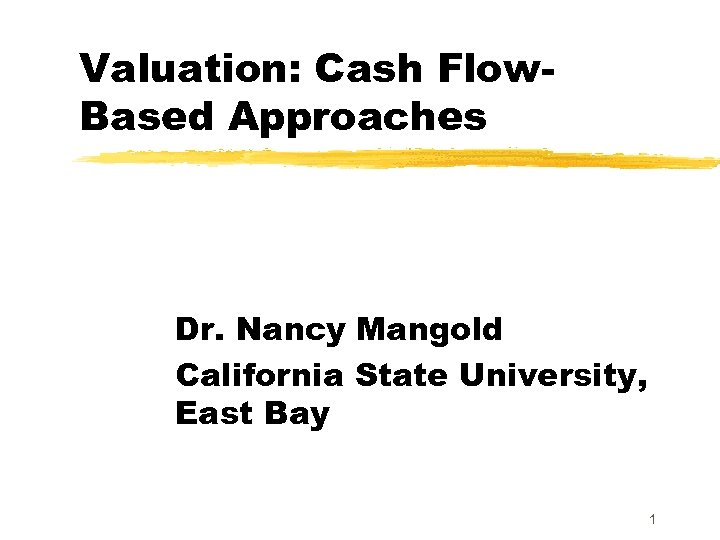Valuation: Cash Flow. Based Approaches Dr. Nancy Mangold California State University, East Bay 1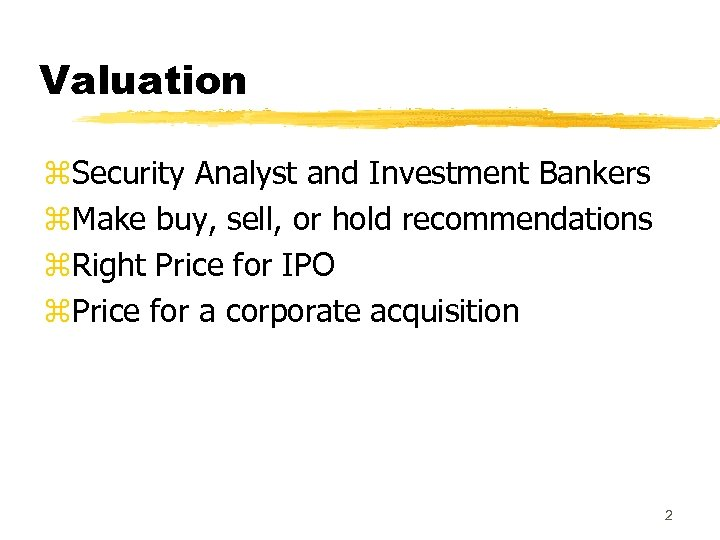Valuation z. Security Analyst and Investment Bankers z. Make buy, sell, or hold recommendations z. Right Price for IPO z. Price for a corporate acquisition 2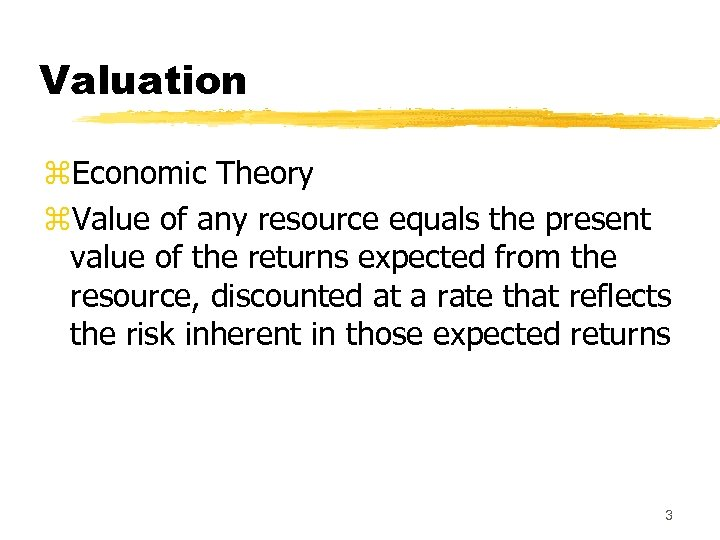Valuation z. Economic Theory z. Value of any resource equals the present value of the returns expected from the resource, discounted at a rate that reflects the risk inherent in those expected returns 3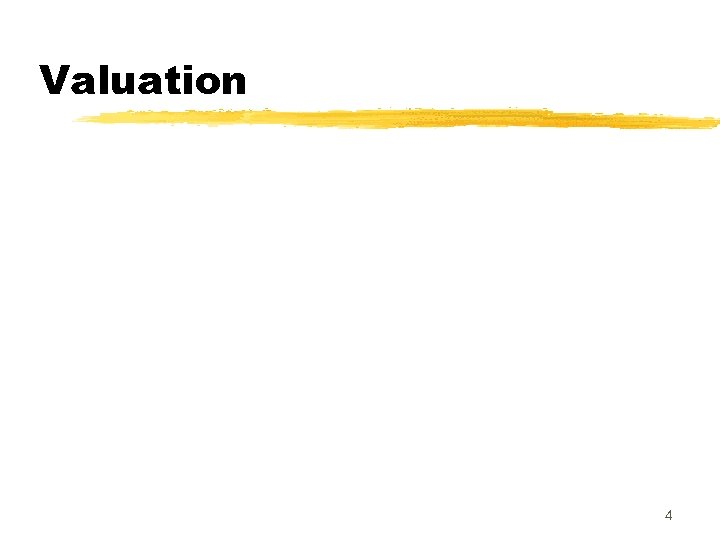Valuation 4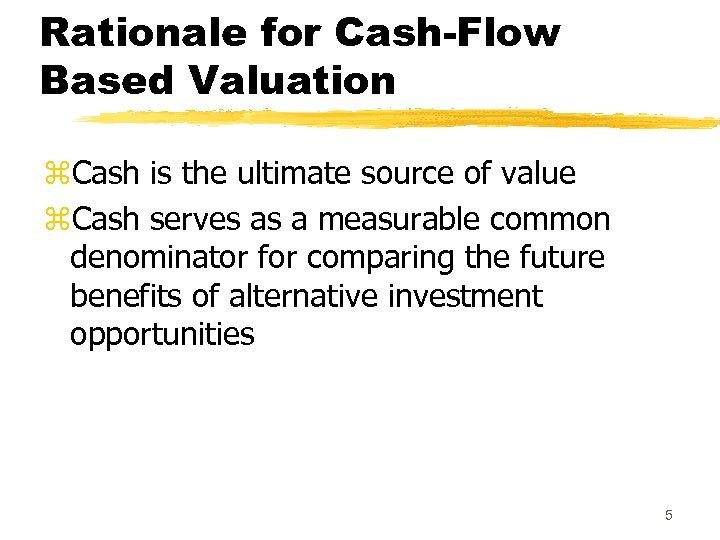Rationale for Cash-Flow Based Valuation z. Cash is the ultimate source of value z. Cash serves as a measurable common denominator for comparing the future benefits of alternative investment opportunities 5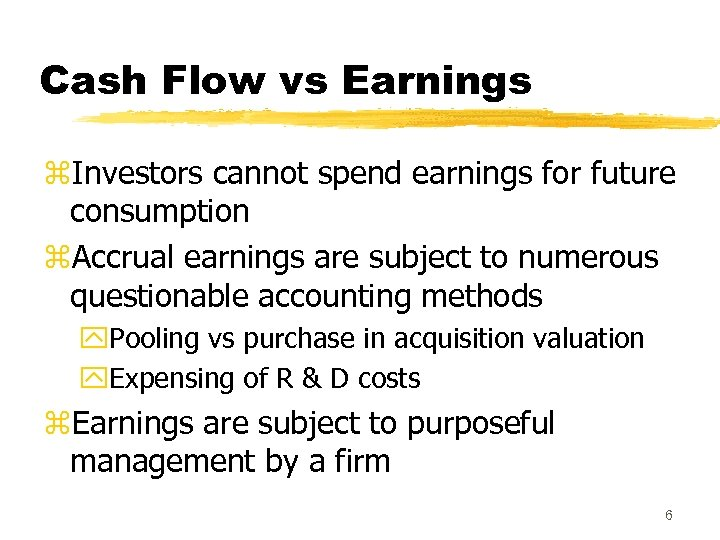Cash Flow vs Earnings z. Investors cannot spend earnings for future consumption z. Accrual earnings are subject to numerous questionable accounting methods y. Pooling vs purchase in acquisition valuation y. Expensing of R & D costs z. Earnings are subject to purposeful management by a firm 6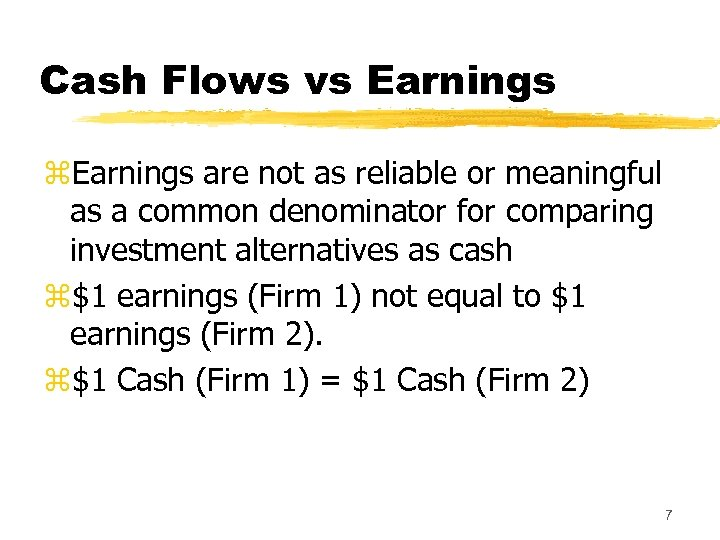Cash Flows vs Earnings z. Earnings are not as reliable or meaningful as a common denominator for comparing investment alternatives as cash z\$1 earnings (Firm 1) not equal to \$1 earnings (Firm 2). z\$1 Cash (Firm 1) = \$1 Cash (Firm 2) 7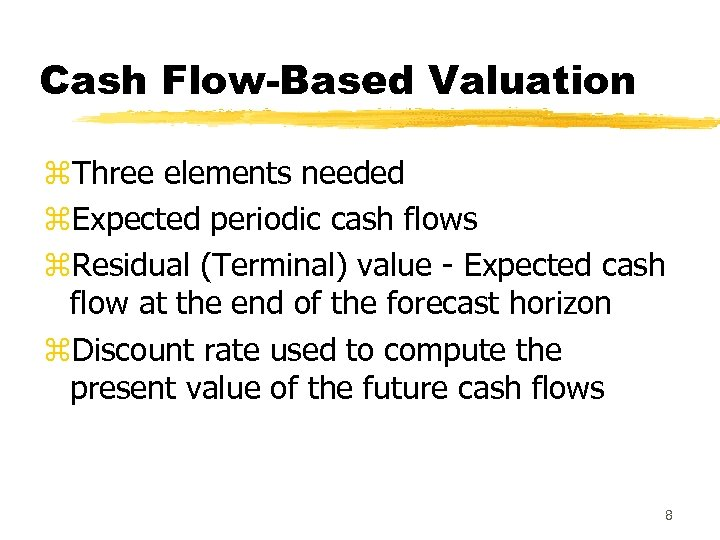Cash Flow-Based Valuation z. Three elements needed z. Expected periodic cash flows z. Residual (Terminal) value - Expected cash flow at the end of the forecast horizon z. Discount rate used to compute the present value of the future cash flows 8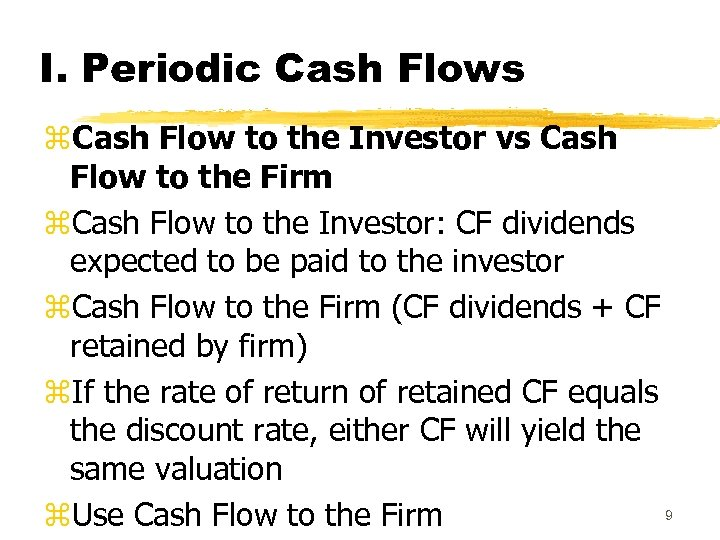I. Periodic Cash Flows z. Cash Flow to the Investor vs Cash Flow to the Firm z. Cash Flow to the Investor: CF dividends expected to be paid to the investor z. Cash Flow to the Firm (CF dividends + CF retained by firm) z. If the rate of return of retained CF equals the discount rate, either CF will yield the same valuation 9 z. Use Cash Flow to the Firm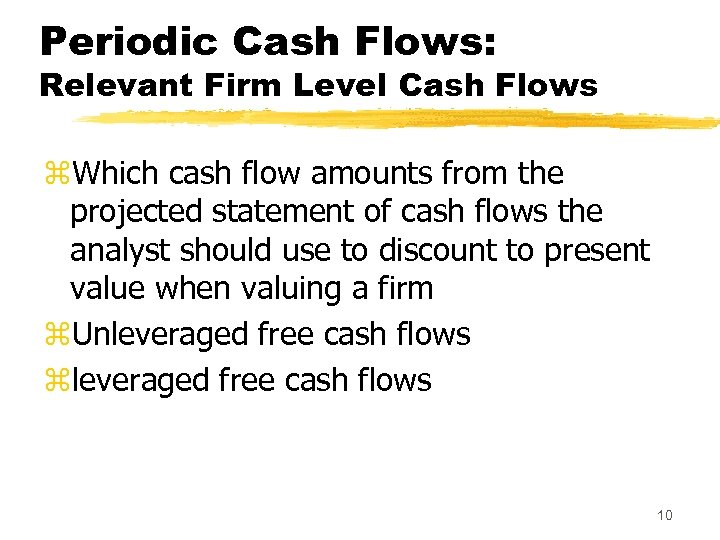Periodic Cash Flows: Relevant Firm Level Cash Flows z. Which cash flow amounts from the projected statement of cash flows the analyst should use to discount to present value when valuing a firm z. Unleveraged free cash flows zleveraged free cash flows 10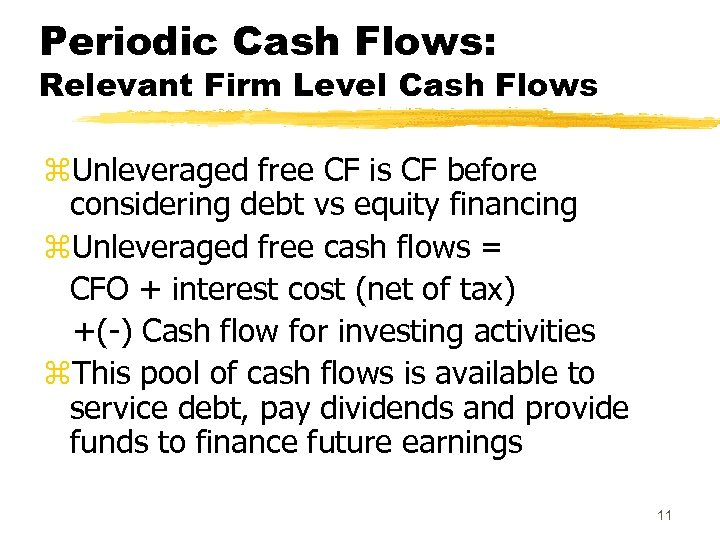Periodic Cash Flows: Relevant Firm Level Cash Flows z. Unleveraged free CF is CF before considering debt vs equity financing z. Unleveraged free cash flows = CFO + interest cost (net of tax) +(-) Cash flow for investing activities z. This pool of cash flows is available to service debt, pay dividends and provide funds to finance future earnings 11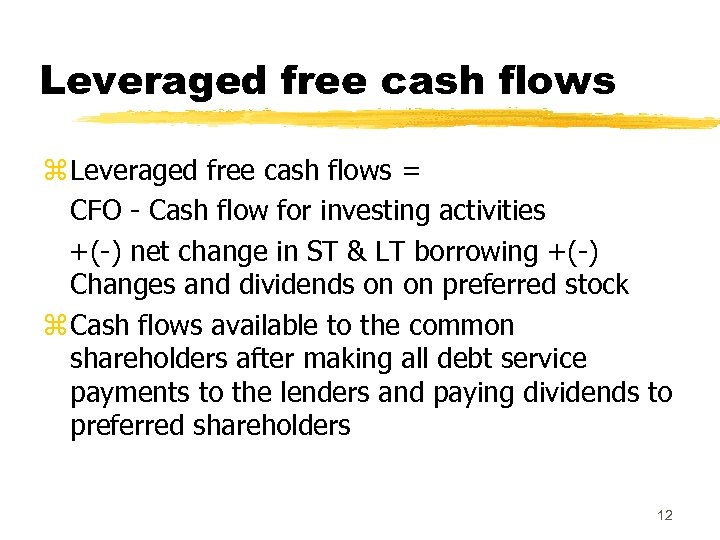Leveraged free cash flows z Leveraged free cash flows = CFO - Cash flow for investing activities +(-) net change in ST & LT borrowing +(-) Changes and dividends on on preferred stock z Cash flows available to the common shareholders after making all debt service payments to the lenders and paying dividends to preferred shareholders 12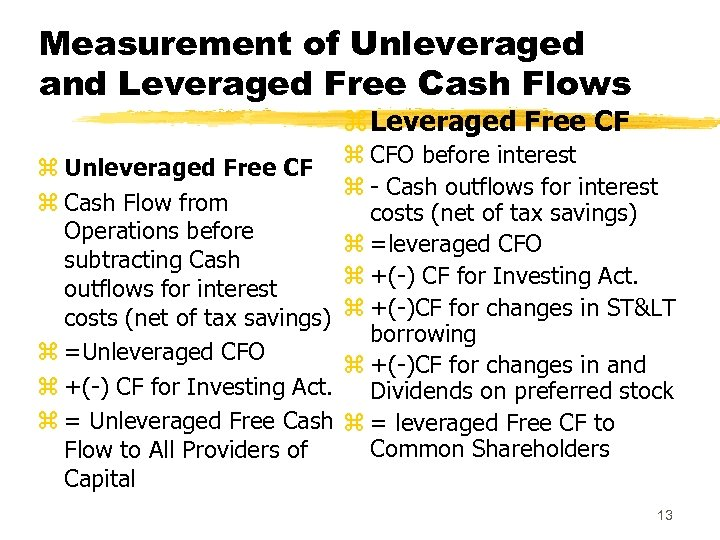Measurement of Unleveraged and Leveraged Free Cash Flows z Leveraged Free CF z Unleveraged Free CF z Cash Flow from Operations before subtracting Cash outflows for interest costs (net of tax savings) z =Unleveraged CFO z +(-) CF for Investing Act. z = Unleveraged Free Cash Flow to All Providers of Capital z CFO before interest z - Cash outflows for interest costs (net of tax savings) z =leveraged CFO z +(-) CF for Investing Act. z +(-)CF for changes in ST< borrowing z +(-)CF for changes in and Dividends on preferred stock z = leveraged Free CF to Common Shareholders 13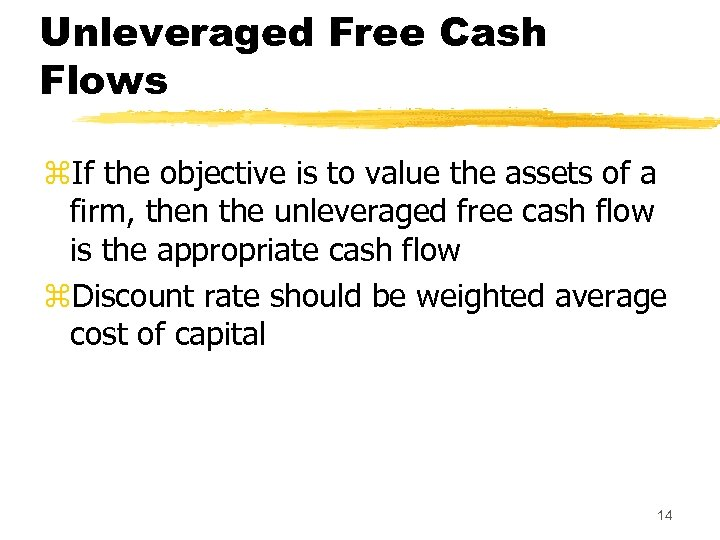Unleveraged Free Cash Flows z. If the objective is to value the assets of a firm, then the unleveraged free cash flow is the appropriate cash flow z. Discount rate should be weighted average cost of capital 14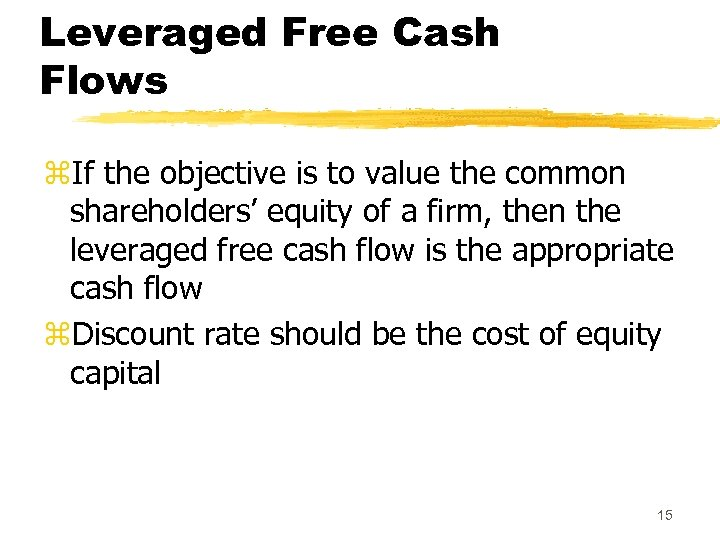Leveraged Free Cash Flows z. If the objective is to value the common shareholders’ equity of a firm, then the leveraged free cash flow is the appropriate cash flow z. Discount rate should be the cost of equity capital 15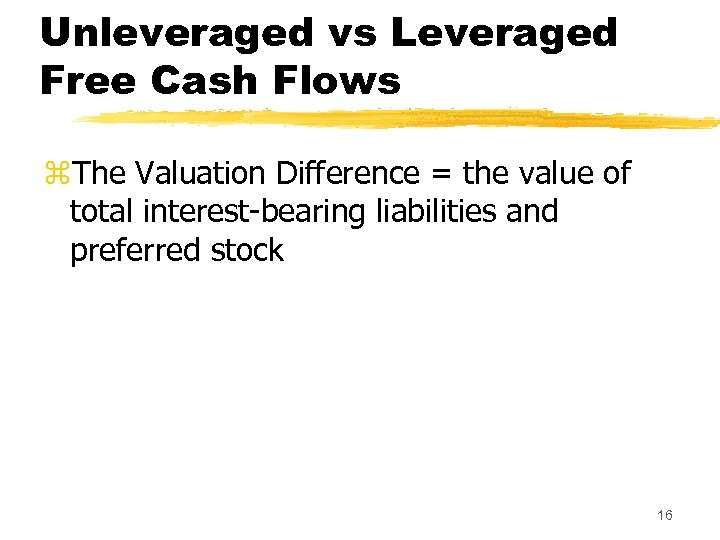Unleveraged vs Leveraged Free Cash Flows z. The Valuation Difference = the value of total interest-bearing liabilities and preferred stock 16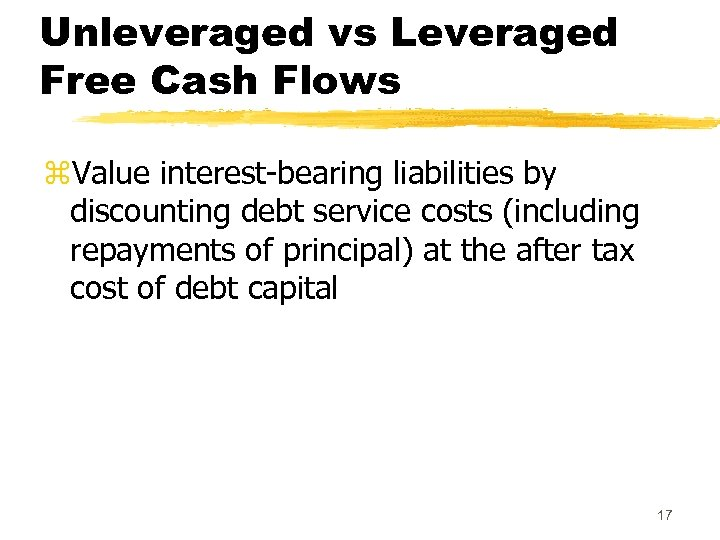Unleveraged vs Leveraged Free Cash Flows z. Value interest-bearing liabilities by discounting debt service costs (including repayments of principal) at the after tax cost of debt capital 17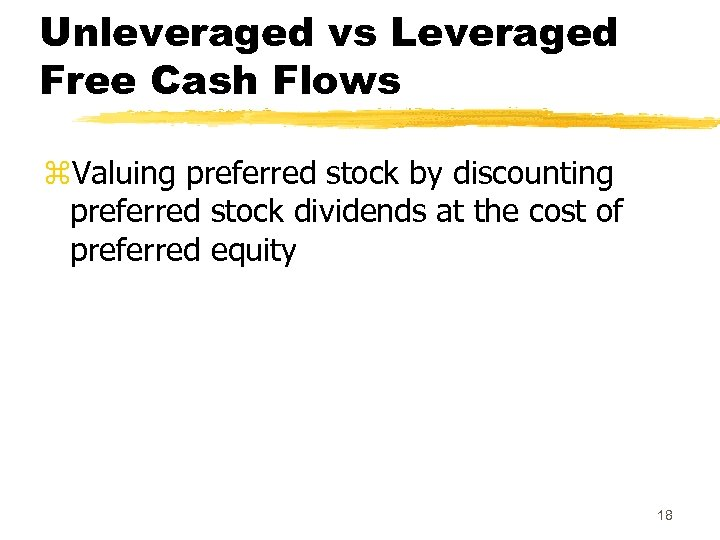Unleveraged vs Leveraged Free Cash Flows z. Valuing preferred stock by discounting preferred stock dividends at the cost of preferred equity 18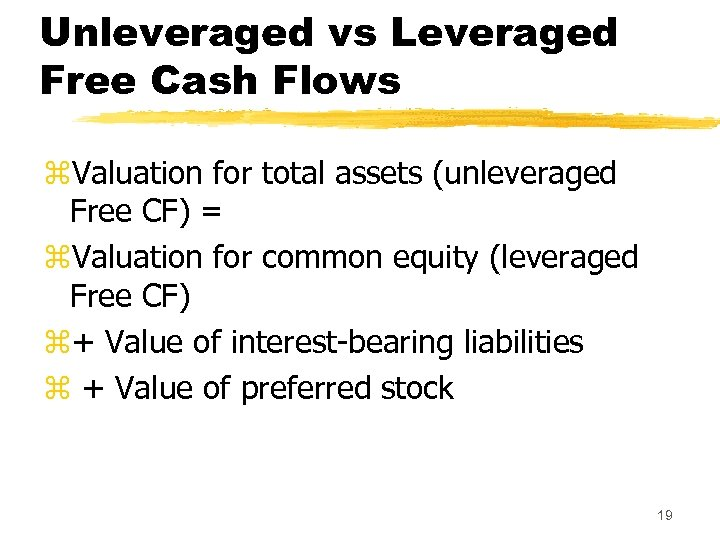Unleveraged vs Leveraged Free Cash Flows z. Valuation for total assets (unleveraged Free CF) = z. Valuation for common equity (leveraged Free CF) z+ Value of interest-bearing liabilities z + Value of preferred stock 19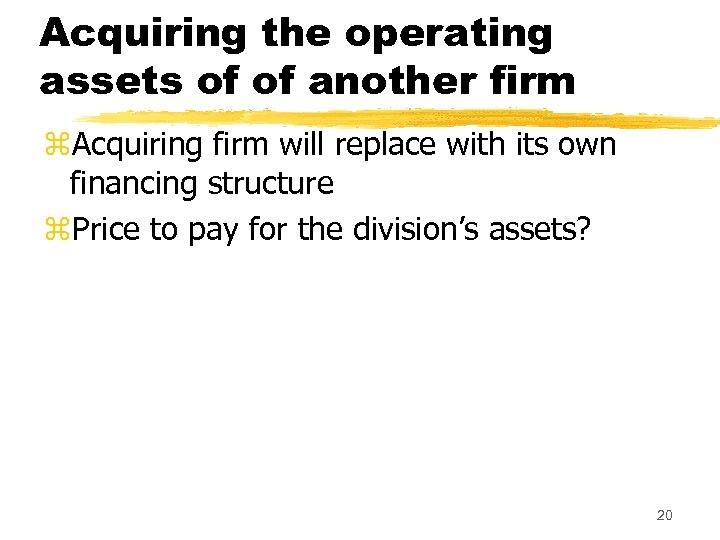Acquiring the operating assets of of another firm z. Acquiring firm will replace with its own financing structure z. Price to pay for the division’s assets? 20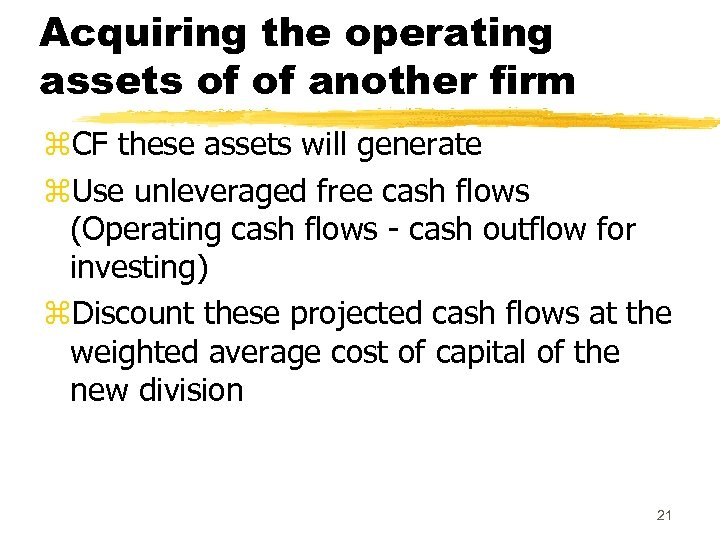Acquiring the operating assets of of another firm z. CF these assets will generate z. Use unleveraged free cash flows (Operating cash flows - cash outflow for investing) z. Discount these projected cash flows at the weighted average cost of capital of the new division 21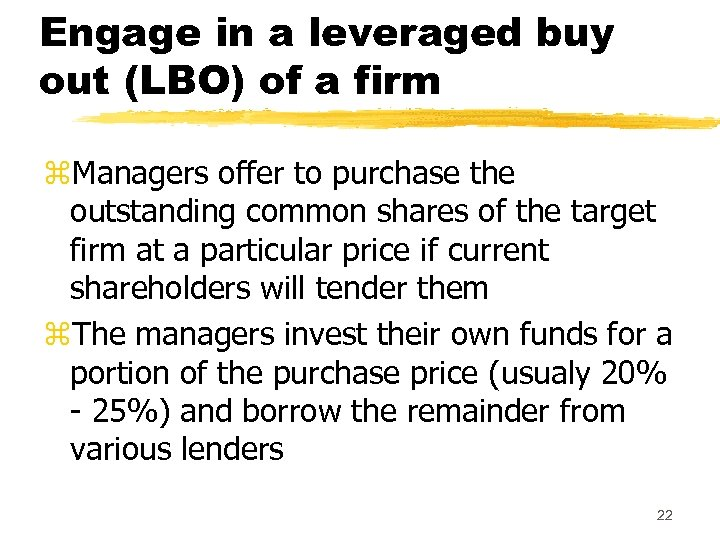Engage in a leveraged buy out (LBO) of a firm z. Managers offer to purchase the outstanding common shares of the target firm at a particular price if current shareholders will tender them z. The managers invest their own funds for a portion of the purchase price (usualy 20% - 25%) and borrow the remainder from various lenders 22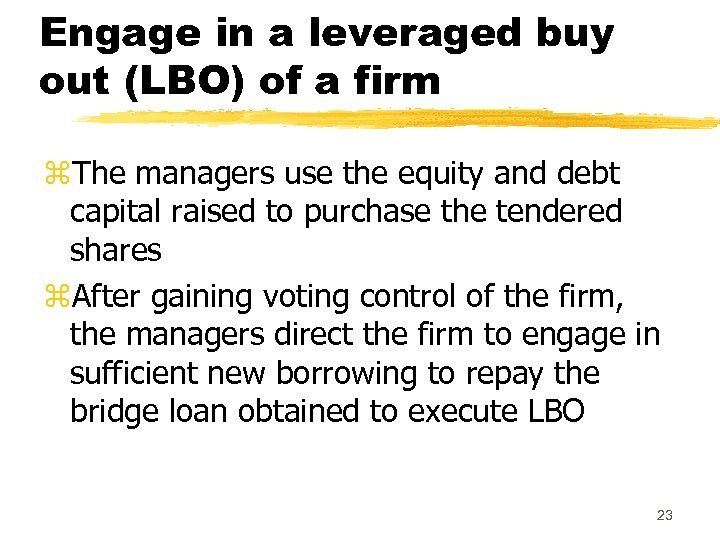Engage in a leveraged buy out (LBO) of a firm z. The managers use the equity and debt capital raised to purchase the tendered shares z. After gaining voting control of the firm, the managers direct the firm to engage in sufficient new borrowing to repay the bridge loan obtained to execute LBO 23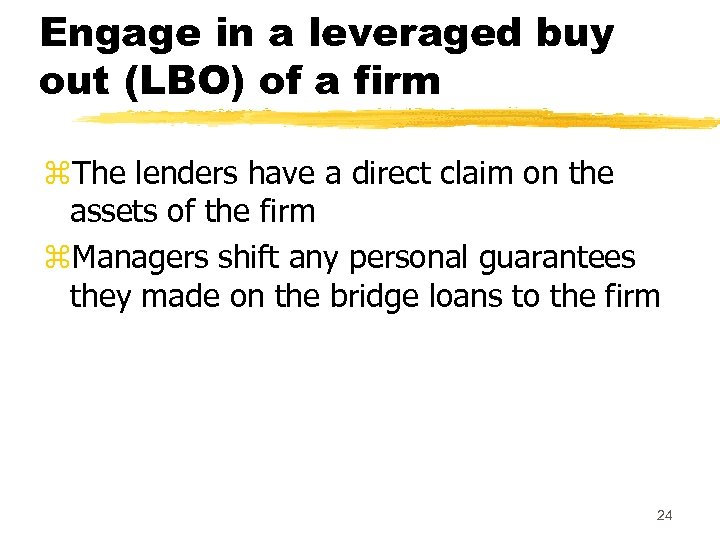Engage in a leveraged buy out (LBO) of a firm z. The lenders have a direct claim on the assets of the firm z. Managers shift any personal guarantees they made on the bridge loans to the firm 24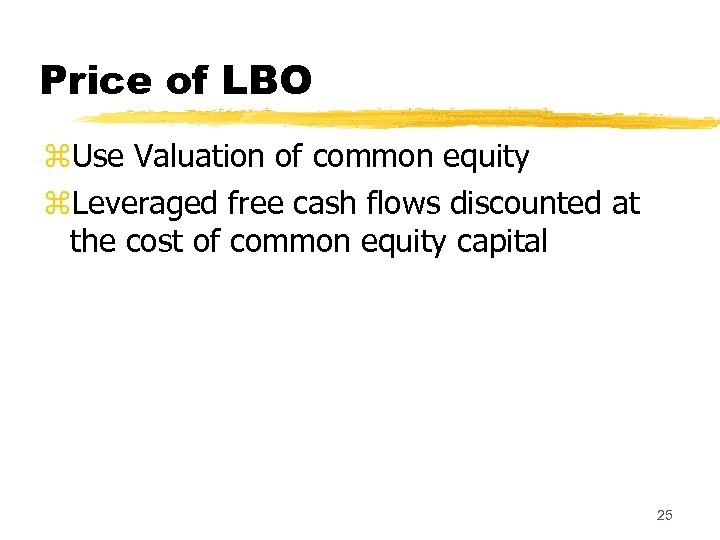Price of LBO z. Use Valuation of common equity z. Leveraged free cash flows discounted at the cost of common equity capital 25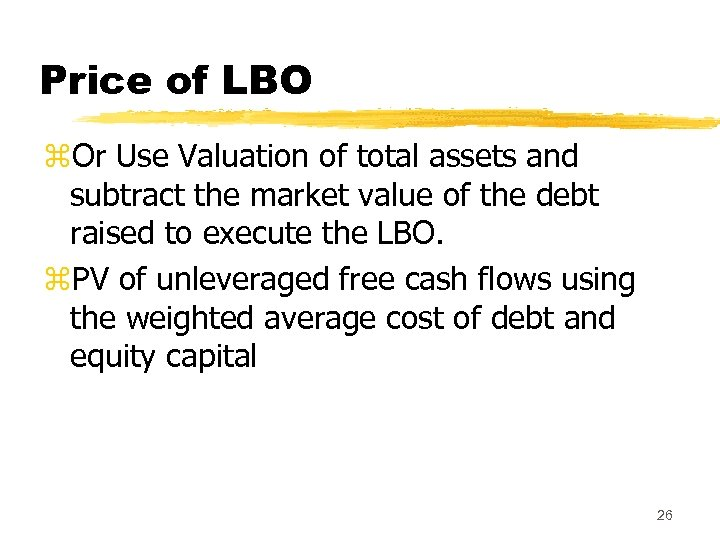Price of LBO z. Or Use Valuation of total assets and subtract the market value of the debt raised to execute the LBO. z. PV of unleveraged free cash flows using the weighted average cost of debt and equity capital 26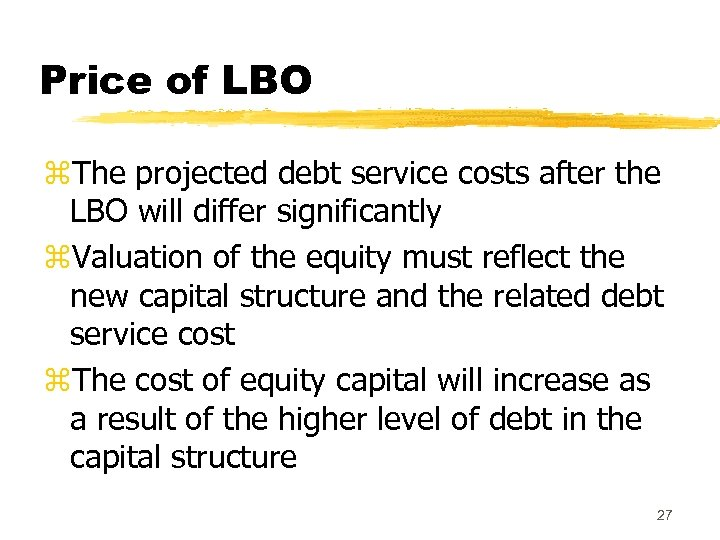Price of LBO z. The projected debt service costs after the LBO will differ significantly z. Valuation of the equity must reflect the new capital structure and the related debt service cost z. The cost of equity capital will increase as a result of the higher level of debt in the capital structure 27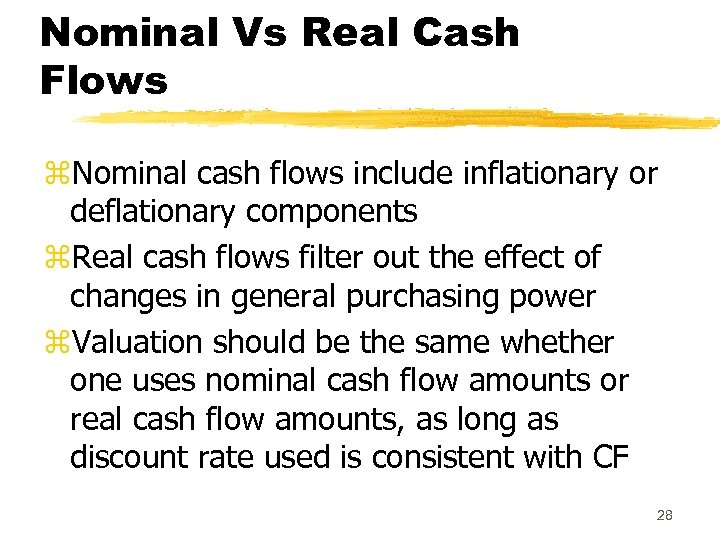Nominal Vs Real Cash Flows z. Nominal cash flows include inflationary or deflationary components z. Real cash flows filter out the effect of changes in general purchasing power z. Valuation should be the same whether one uses nominal cash flow amounts or real cash flow amounts, as long as discount rate used is consistent with CF 28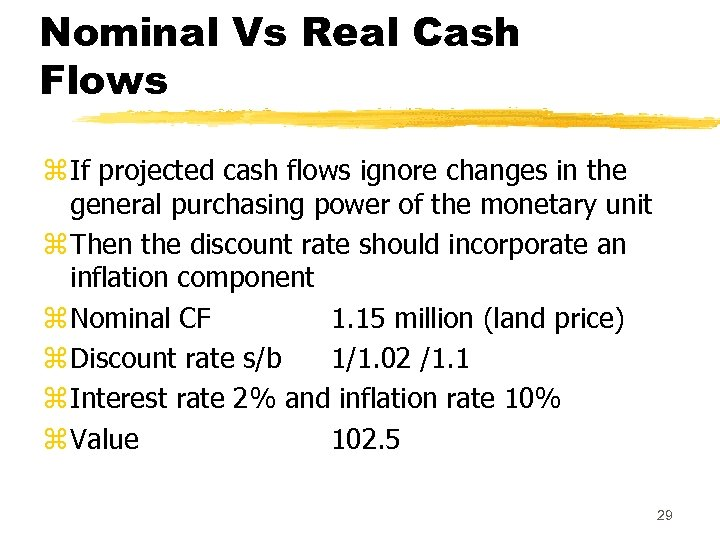Nominal Vs Real Cash Flows z If projected cash flows ignore changes in the general purchasing power of the monetary unit z Then the discount rate should incorporate an inflation component z Nominal CF 1. 15 million (land price) z Discount rate s/b 1/1. 02 /1. 1 z Interest rate 2% and inflation rate 10% z Value 102. 5 29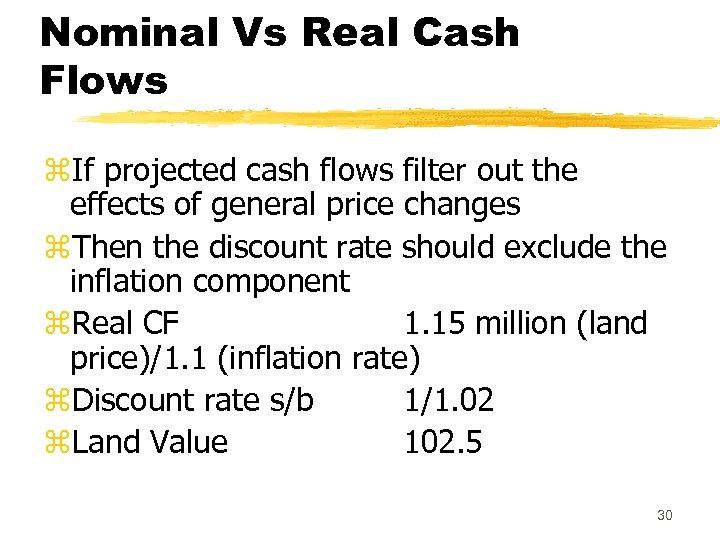Nominal Vs Real Cash Flows z. If projected cash flows filter out the effects of general price changes z. Then the discount rate should exclude the inflation component z. Real CF 1. 15 million (land price)/1. 1 (inflation rate) z. Discount rate s/b 1/1. 02 z. Land Value 102. 5 30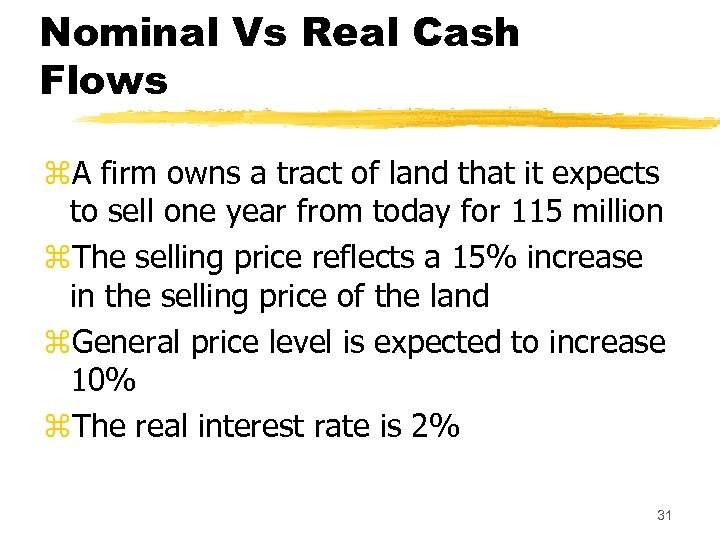Nominal Vs Real Cash Flows z. A firm owns a tract of land that it expects to sell one year from today for 115 million z. The selling price reflects a 15% increase in the selling price of the land z. General price level is expected to increase 10% z. The real interest rate is 2% 31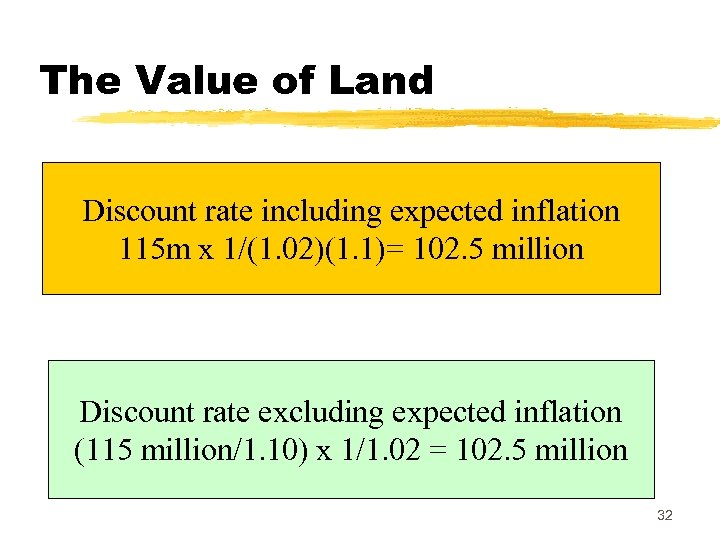The Value of Land Discount rate including expected inflation 115 m x 1/(1. 02)(1. 1)= 102. 5 million Discount rate excluding expected inflation (115 million/1. 10) x 1/1. 02 = 102. 5 million 32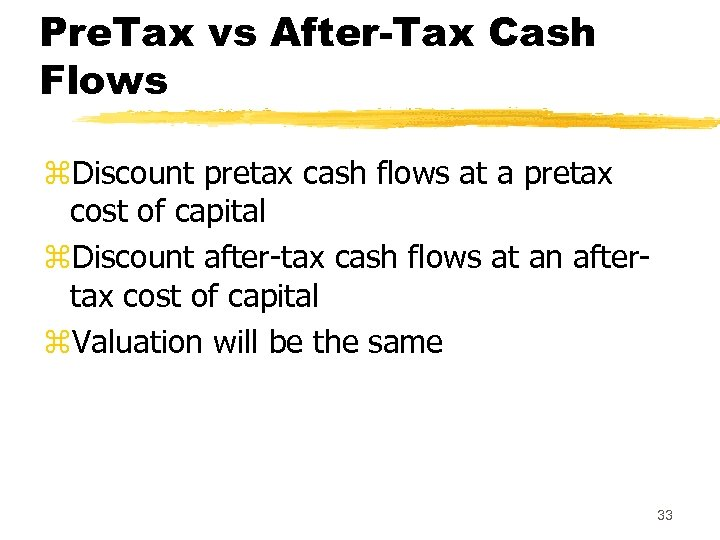Pre. Tax vs After-Tax Cash Flows z. Discount pretax cash flows at a pretax cost of capital z. Discount after-tax cash flows at an aftertax cost of capital z. Valuation will be the same 33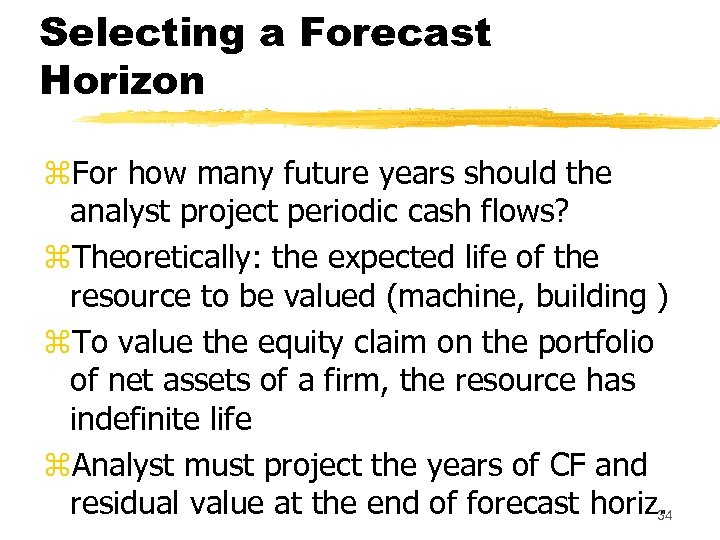Selecting a Forecast Horizon z. For how many future years should the analyst project periodic cash flows? z. Theoretically: the expected life of the resource to be valued (machine, building ) z. To value the equity claim on the portfolio of net assets of a firm, the resource has indefinite life z. Analyst must project the years of CF and residual value at the end of forecast horiz. 4 3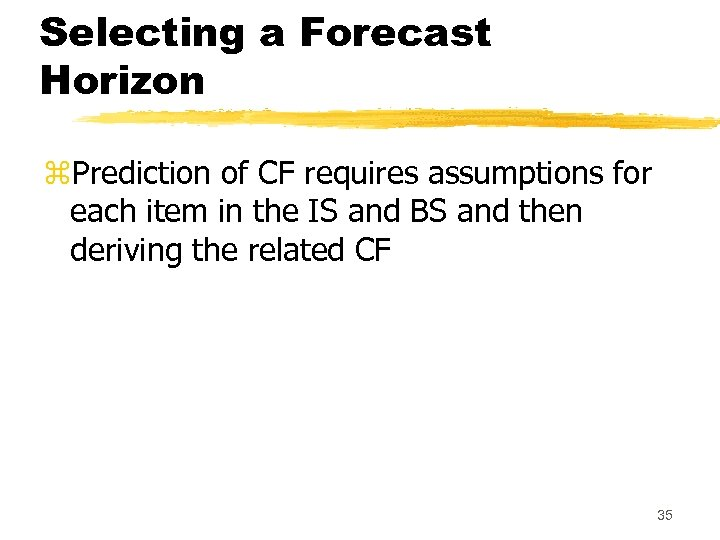Selecting a Forecast Horizon z. Prediction of CF requires assumptions for each item in the IS and BS and then deriving the related CF 35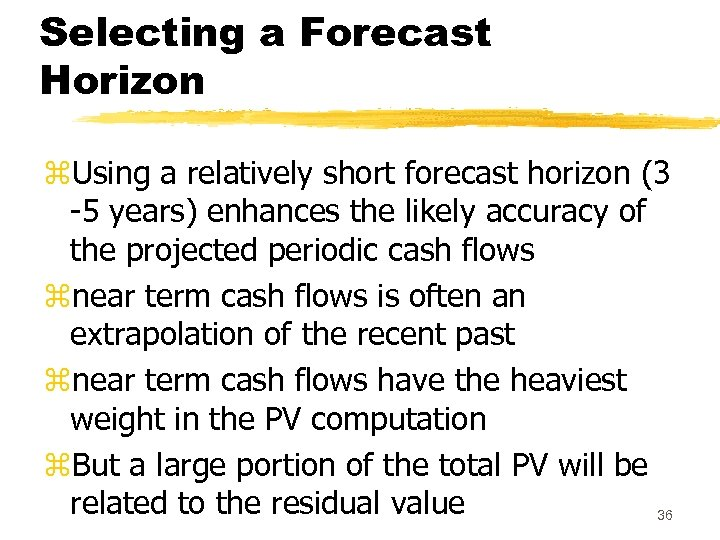Selecting a Forecast Horizon z. Using a relatively short forecast horizon (3 -5 years) enhances the likely accuracy of the projected periodic cash flows znear term cash flows is often an extrapolation of the recent past znear term cash flows have the heaviest weight in the PV computation z. But a large portion of the total PV will be related to the residual value 36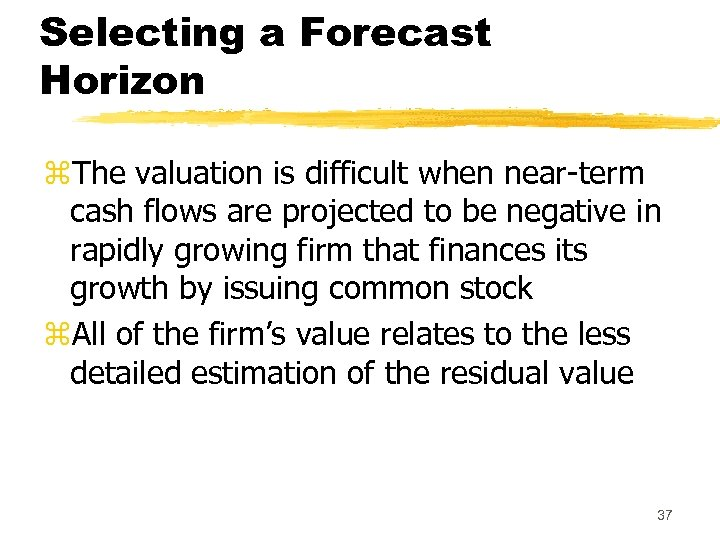Selecting a Forecast Horizon z. The valuation is difficult when near-term cash flows are projected to be negative in rapidly growing firm that finances its growth by issuing common stock z. All of the firm’s value relates to the less detailed estimation of the residual value 37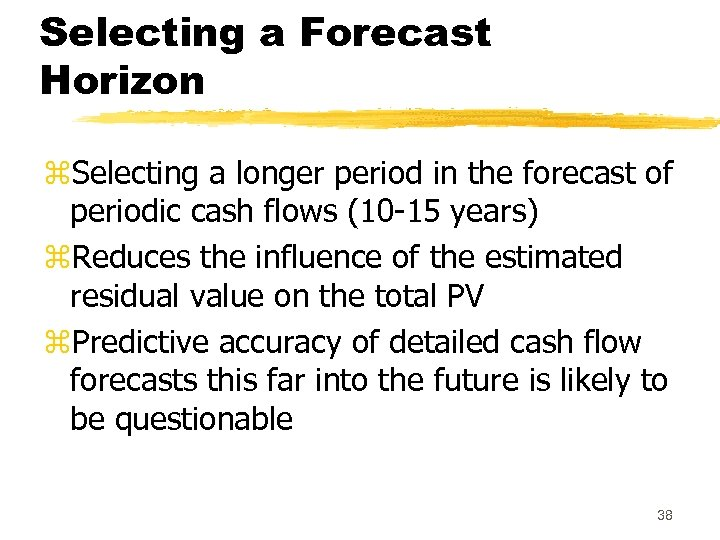Selecting a Forecast Horizon z. Selecting a longer period in the forecast of periodic cash flows (10 -15 years) z. Reduces the influence of the estimated residual value on the total PV z. Predictive accuracy of detailed cash flow forecasts this far into the future is likely to be questionable 38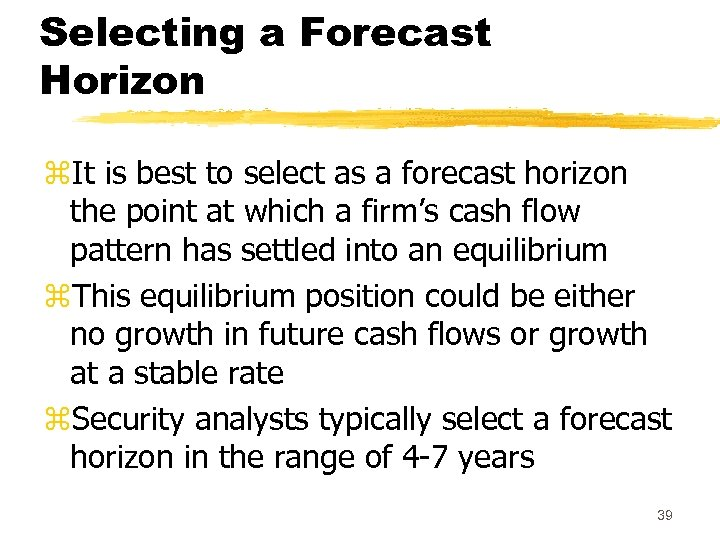Selecting a Forecast Horizon z. It is best to select as a forecast horizon the point at which a firm’s cash flow pattern has settled into an equilibrium z. This equilibrium position could be either no growth in future cash flows or growth at a stable rate z. Security analysts typically select a forecast horizon in the range of 4 -7 years 39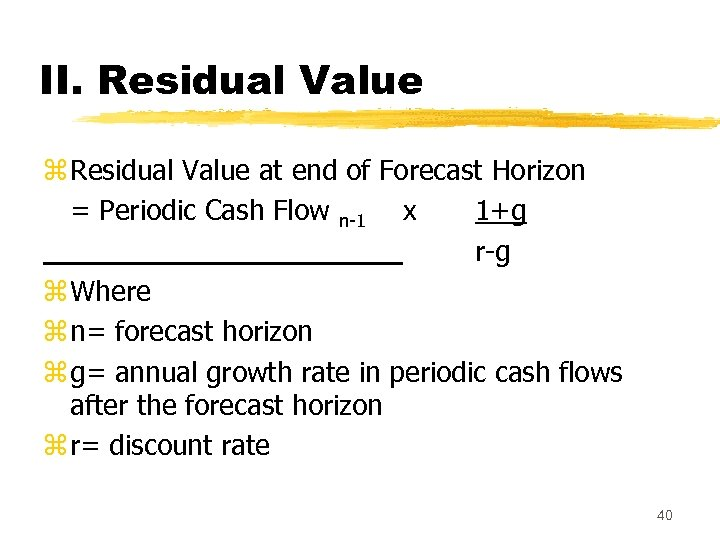II. Residual Value z Residual Value at end of Forecast Horizon = Periodic Cash Flow n-1 x 1+g r-g z Where z n= forecast horizon z g= annual growth rate in periodic cash flows after the forecast horizon z r= discount rate 40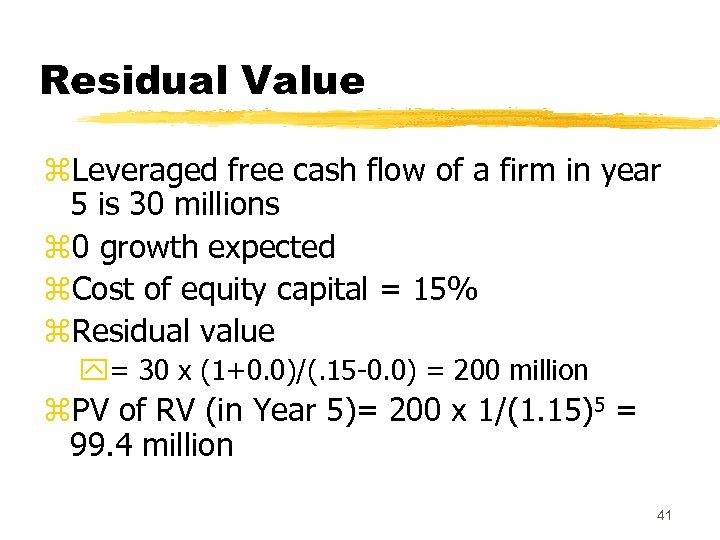Residual Value z. Leveraged free cash flow of a firm in year 5 is 30 millions z 0 growth expected z. Cost of equity capital = 15% z. Residual value y= 30 x (1+0. 0)/(. 15 -0. 0) = 200 million z. PV of RV (in Year 5)= 200 x 1/(1. 15)5 = 99. 4 million 41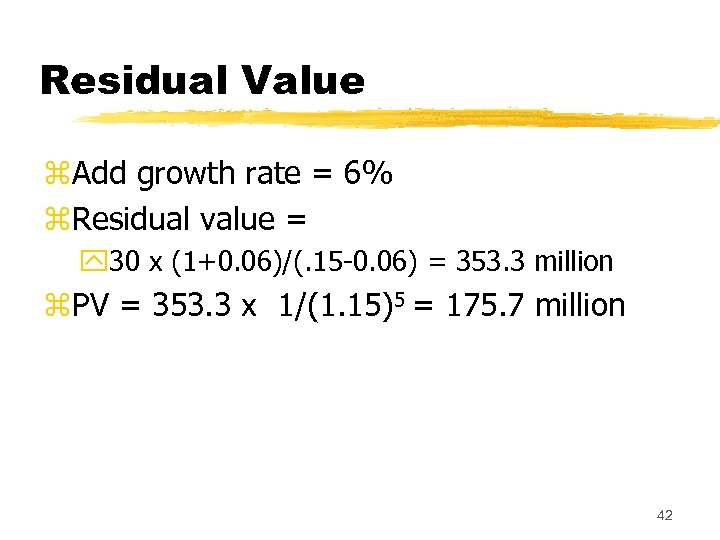Residual Value z. Add growth rate = 6% z. Residual value = y 30 x (1+0. 06)/(. 15 -0. 06) = 353. 3 million z. PV = 353. 3 x 1/(1. 15)5 = 175. 7 million 42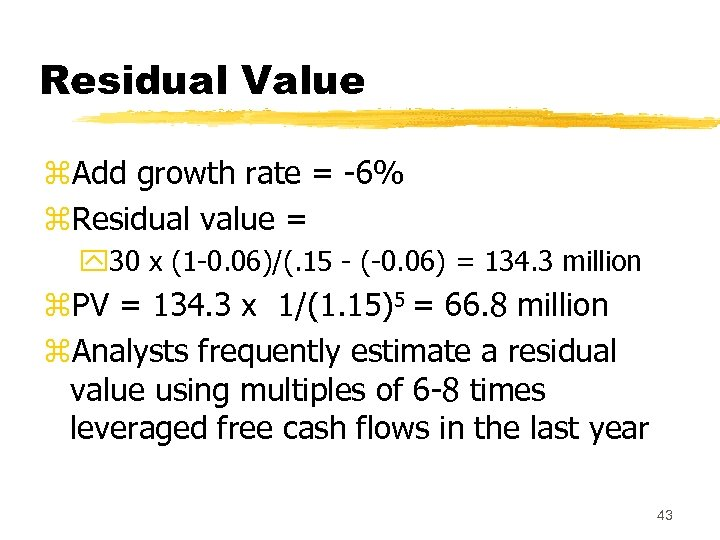Residual Value z. Add growth rate = -6% z. Residual value = y 30 x (1 -0. 06)/(. 15 - (-0. 06) = 134. 3 million z. PV = 134. 3 x 1/(1. 15)5 = 66. 8 million z. Analysts frequently estimate a residual value using multiples of 6 -8 times leveraged free cash flows in the last year 43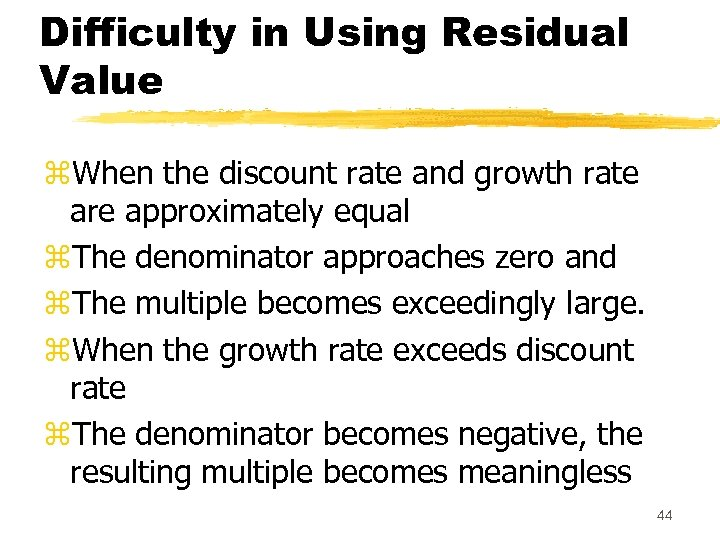Difficulty in Using Residual Value z. When the discount rate and growth rate are approximately equal z. The denominator approaches zero and z. The multiple becomes exceedingly large. z. When the growth rate exceeds discount rate z. The denominator becomes negative, the resulting multiple becomes meaningless 44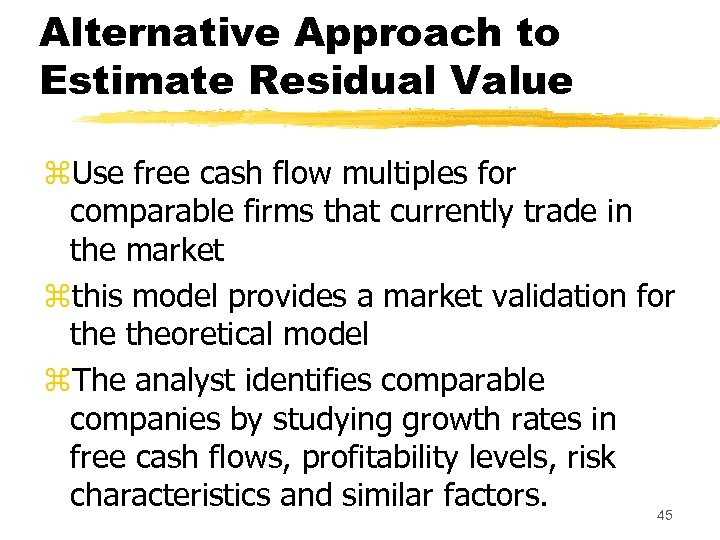Alternative Approach to Estimate Residual Value z. Use free cash flow multiples for comparable firms that currently trade in the market zthis model provides a market validation for theoretical model z. The analyst identifies comparable companies by studying growth rates in free cash flows, profitability levels, risk characteristics and similar factors. 45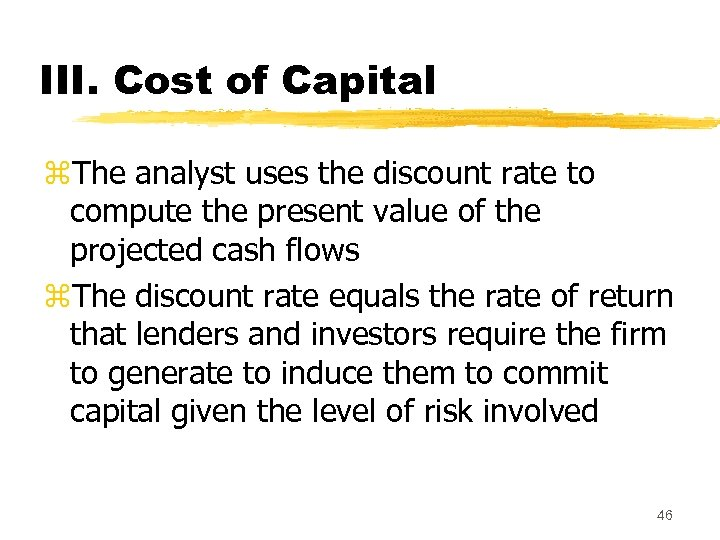III. Cost of Capital z. The analyst uses the discount rate to compute the present value of the projected cash flows z. The discount rate equals the rate of return that lenders and investors require the firm to generate to induce them to commit capital given the level of risk involved 46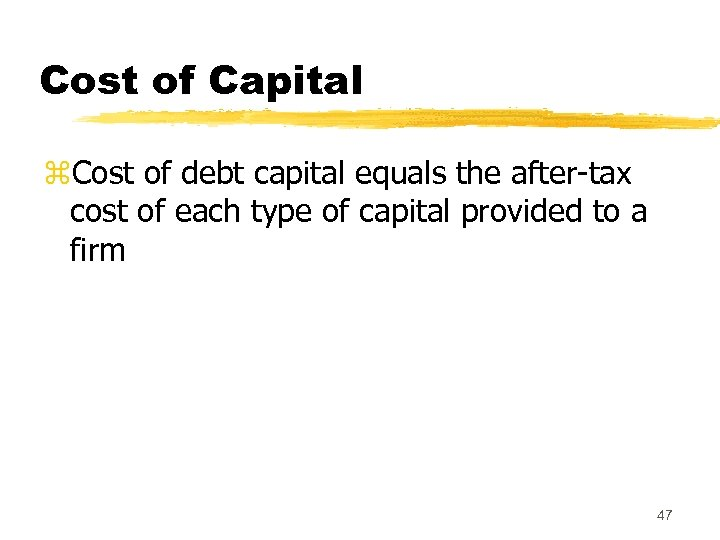Cost of Capital z. Cost of debt capital equals the after-tax cost of each type of capital provided to a firm 47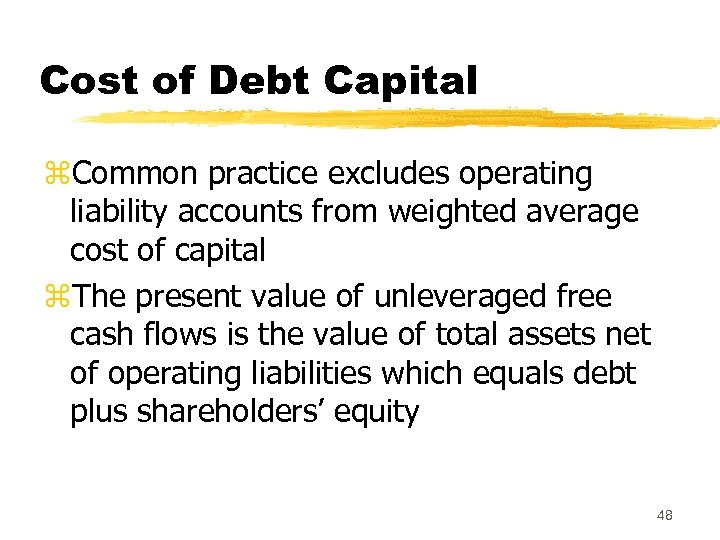Cost of Debt Capital z. Common practice excludes operating liability accounts from weighted average cost of capital z. The present value of unleveraged free cash flows is the value of total assets net of operating liabilities which equals debt plus shareholders’ equity 48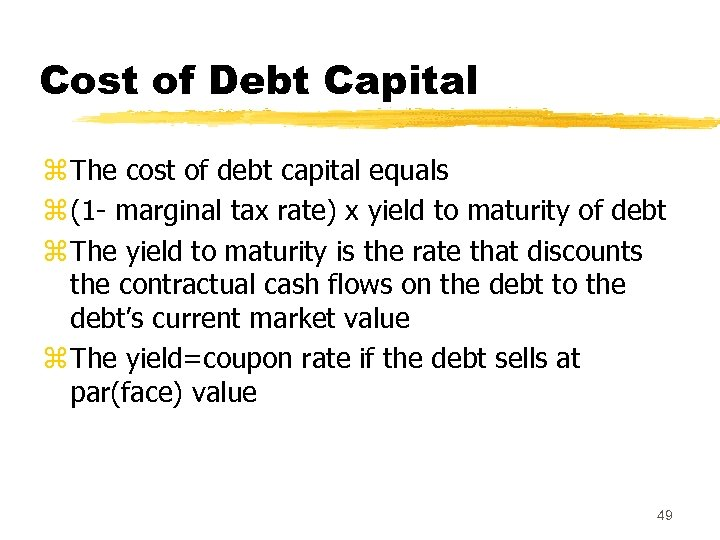Cost of Debt Capital z The cost of debt capital equals z (1 - marginal tax rate) x yield to maturity of debt z The yield to maturity is the rate that discounts the contractual cash flows on the debt to the debt’s current market value z The yield=coupon rate if the debt sells at par(face) value 49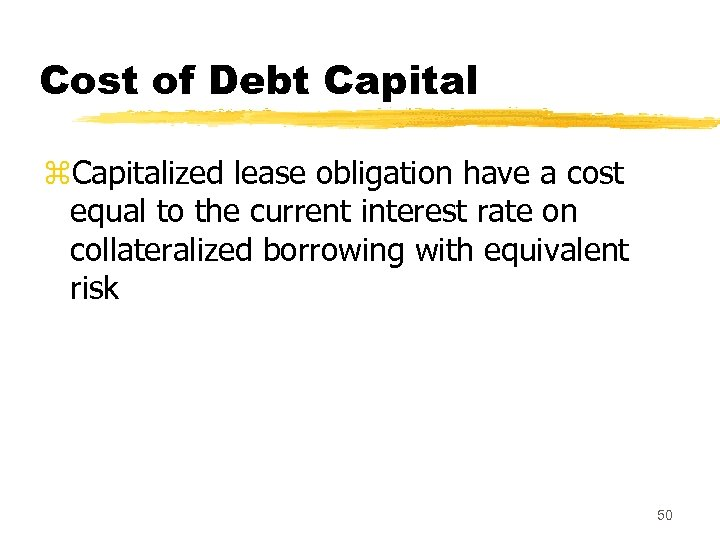Cost of Debt Capital z. Capitalized lease obligation have a cost equal to the current interest rate on collateralized borrowing with equivalent risk 50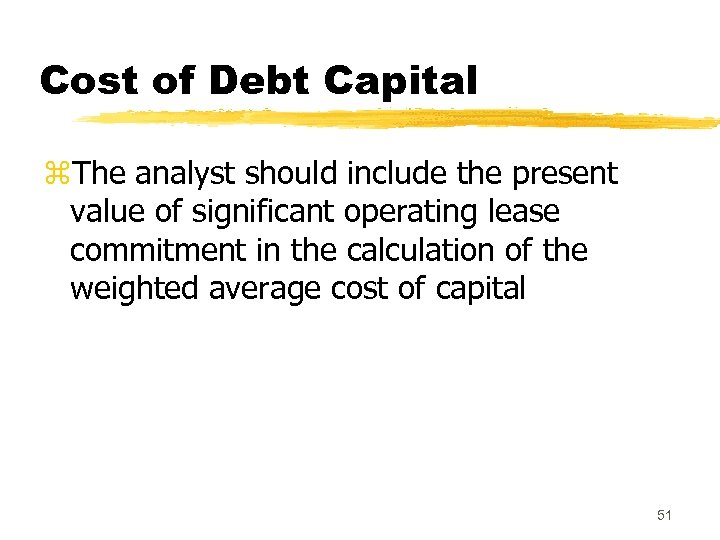Cost of Debt Capital z. The analyst should include the present value of significant operating lease commitment in the calculation of the weighted average cost of capital 51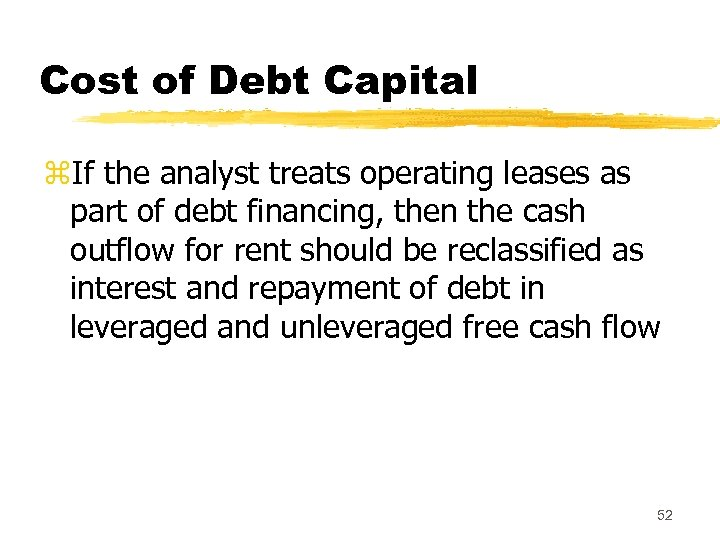Cost of Debt Capital z. If the analyst treats operating leases as part of debt financing, then the cash outflow for rent should be reclassified as interest and repayment of debt in leveraged and unleveraged free cash flow 52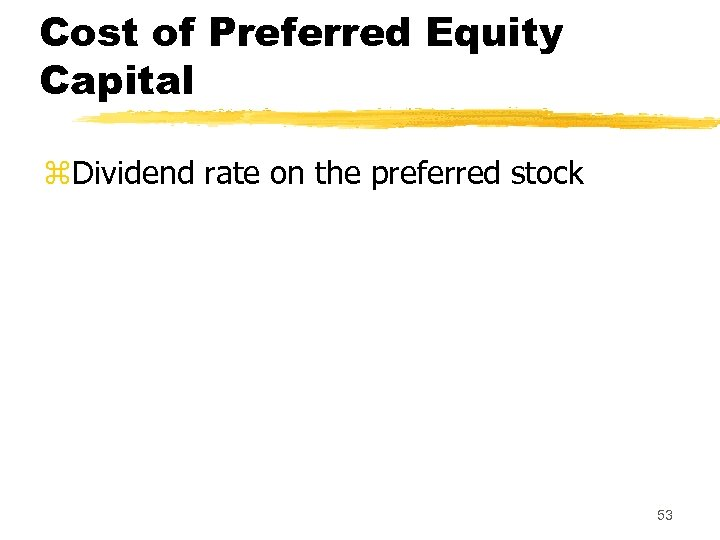Cost of Preferred Equity Capital z. Dividend rate on the preferred stock 53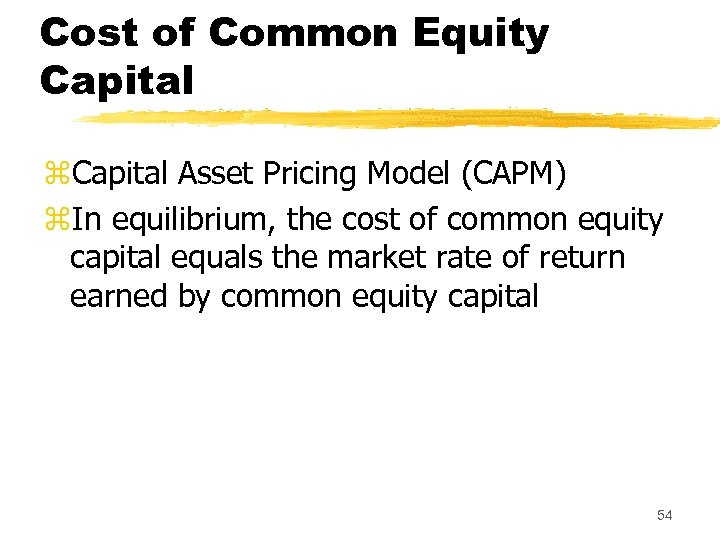Cost of Common Equity Capital z. Capital Asset Pricing Model (CAPM) z. In equilibrium, the cost of common equity capital equals the market rate of return earned by common equity capital 54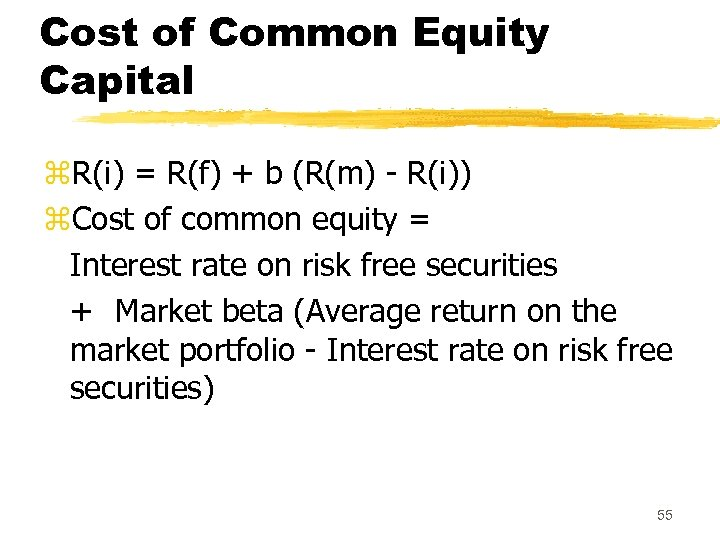Cost of Common Equity Capital z. R(i) = R(f) + b (R(m) - R(i)) z. Cost of common equity = Interest rate on risk free securities + Market beta (Average return on the market portfolio - Interest rate on risk free securities) 55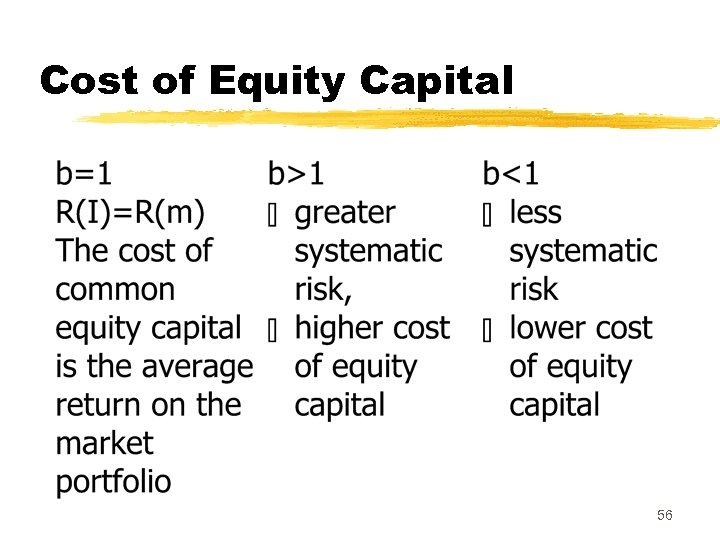Cost of Equity Capital 56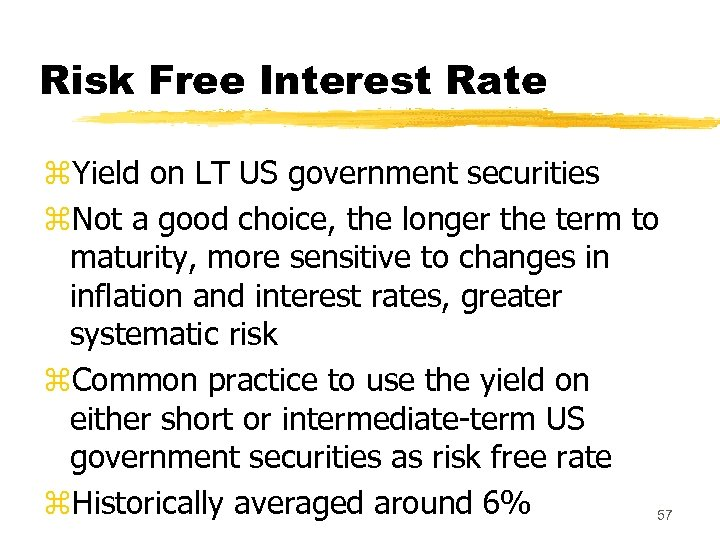Risk Free Interest Rate z. Yield on LT US government securities z. Not a good choice, the longer the term to maturity, more sensitive to changes in inflation and interest rates, greater systematic risk z. Common practice to use the yield on either short or intermediate-term US government securities as risk free rate z. Historically averaged around 6% 57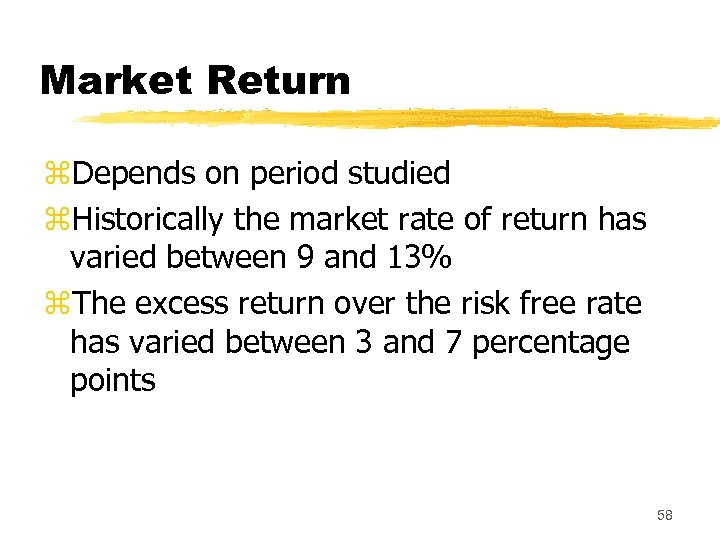Market Return z. Depends on period studied z. Historically the market rate of return has varied between 9 and 13% z. The excess return over the risk free rate has varied between 3 and 7 percentage points 58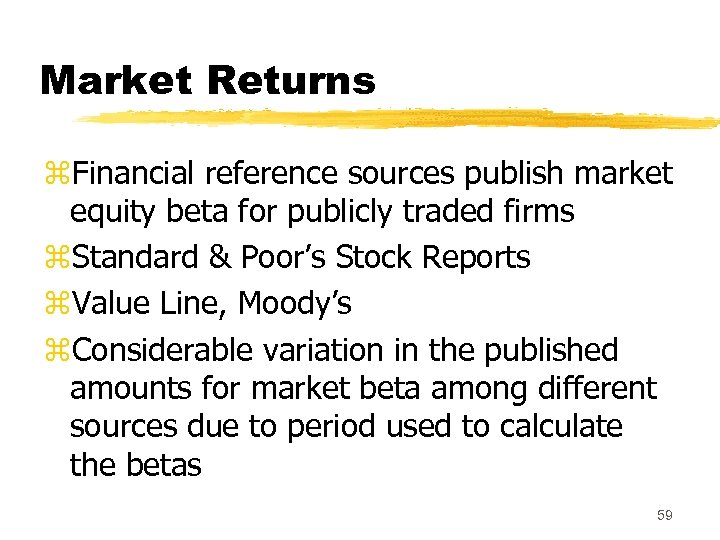Market Returns z. Financial reference sources publish market equity beta for publicly traded firms z. Standard & Poor’s Stock Reports z. Value Line, Moody’s z. Considerable variation in the published amounts for market beta among different sources due to period used to calculate the betas 59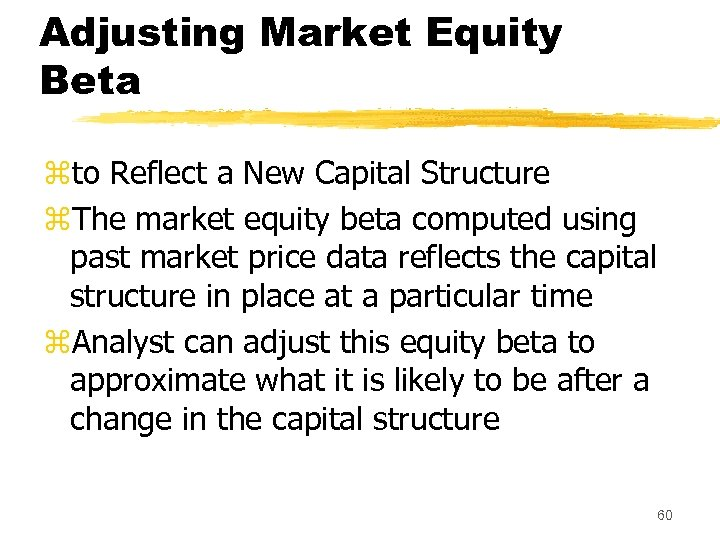Adjusting Market Equity Beta zto Reflect a New Capital Structure z. The market equity beta computed using past market price data reflects the capital structure in place at a particular time z. Analyst can adjust this equity beta to approximate what it is likely to be after a change in the capital structure 60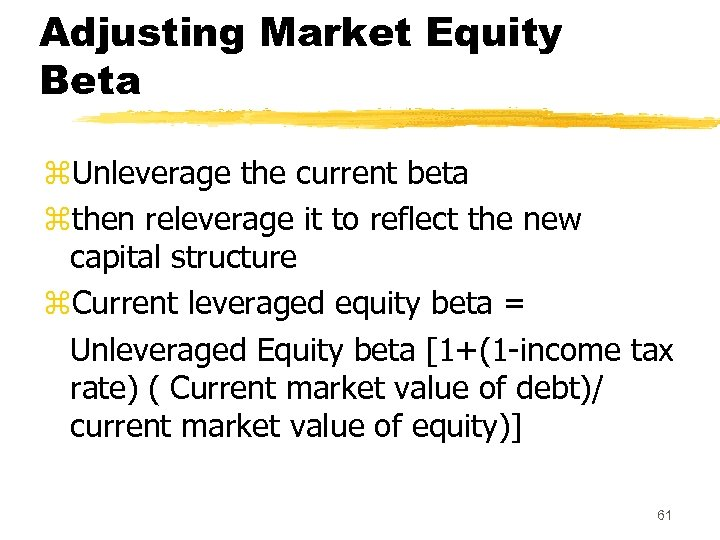Adjusting Market Equity Beta z. Unleverage the current beta zthen releverage it to reflect the new capital structure z. Current leveraged equity beta = Unleveraged Equity beta [1+(1 -income tax rate) ( Current market value of debt)/ current market value of equity)] 61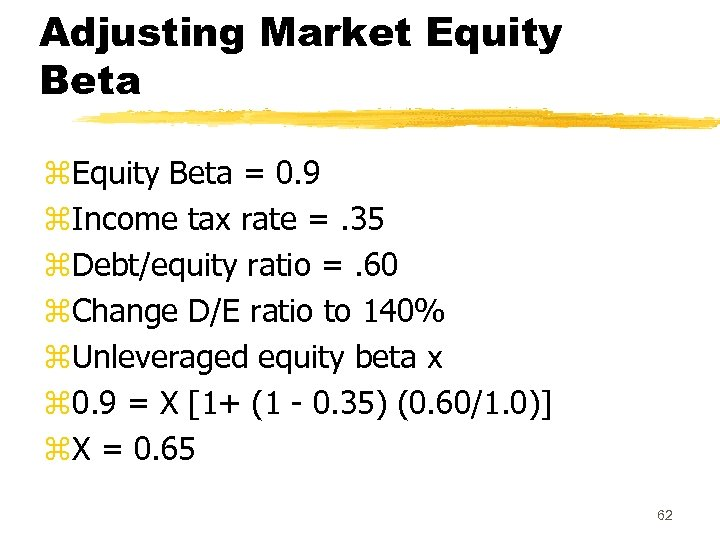Adjusting Market Equity Beta z. Equity Beta = 0. 9 z. Income tax rate =. 35 z. Debt/equity ratio =. 60 z. Change D/E ratio to 140% z. Unleveraged equity beta x z 0. 9 = X [1+ (1 - 0. 35) (0. 60/1. 0)] z. X = 0. 65 62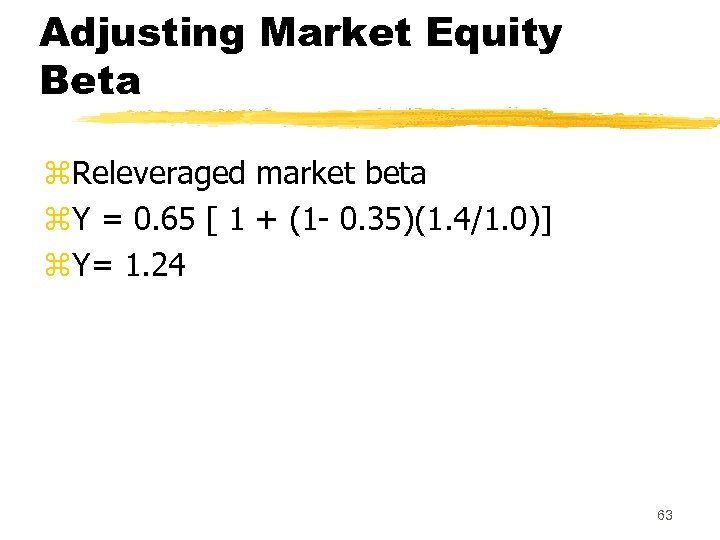Adjusting Market Equity Beta z. Releveraged market beta z. Y = 0. 65 [ 1 + (1 - 0. 35)(1. 4/1. 0)] z. Y= 1. 24 63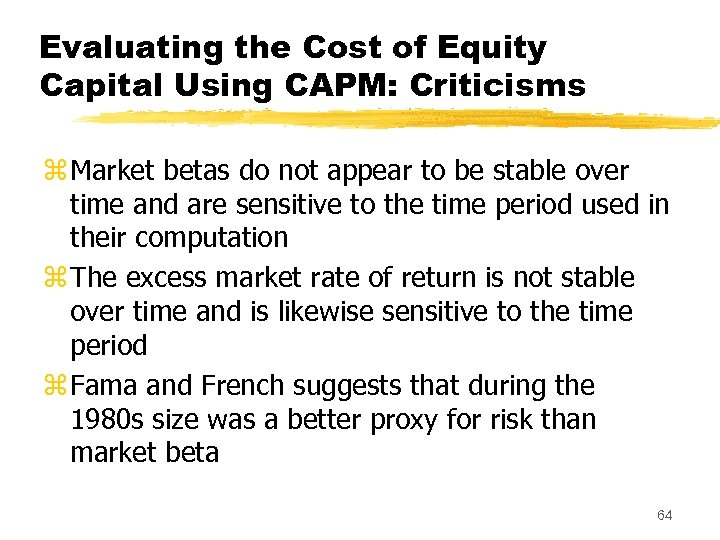Evaluating the Cost of Equity Capital Using CAPM: Criticisms z Market betas do not appear to be stable over time and are sensitive to the time period used in their computation z The excess market rate of return is not stable over time and is likewise sensitive to the time period z Fama and French suggests that during the 1980 s size was a better proxy for risk than market beta 64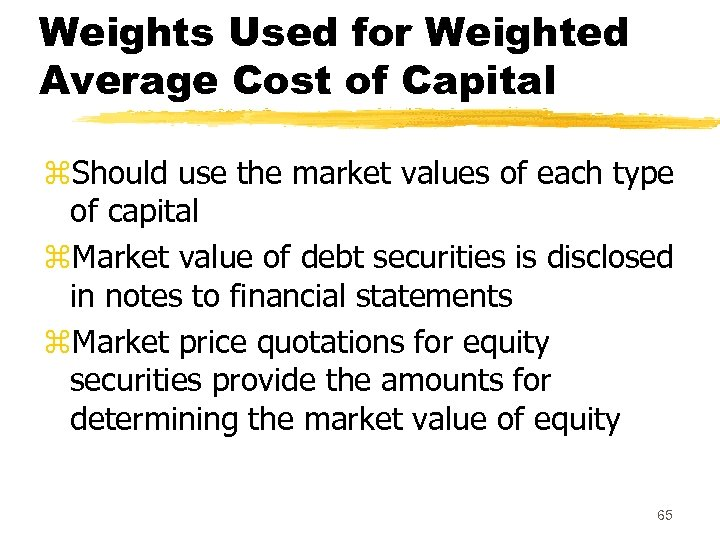Weights Used for Weighted Average Cost of Capital z. Should use the market values of each type of capital z. Market value of debt securities is disclosed in notes to financial statements z. Market price quotations for equity securities provide the amounts for determining the market value of equity 65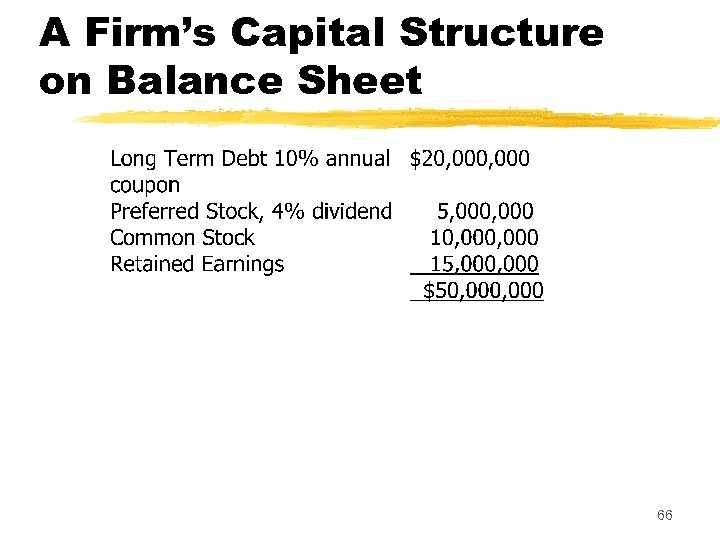A Firm’s Capital Structure on Balance Sheet 66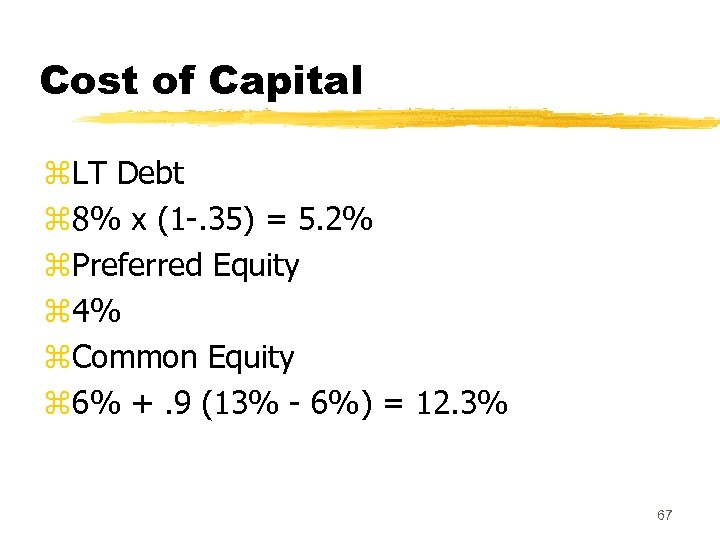Cost of Capital z. LT Debt z 8% x (1 -. 35) = 5. 2% z. Preferred Equity z 4% z. Common Equity z 6% +. 9 (13% - 6%) = 12. 3% 67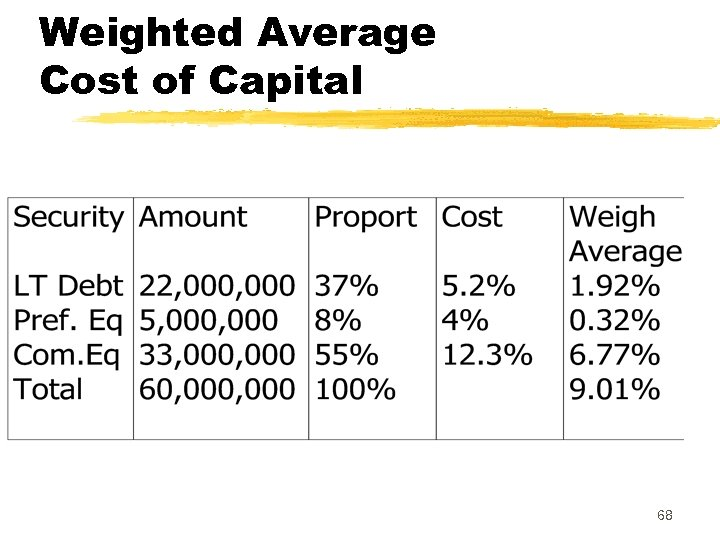Weighted Average Cost of Capital 68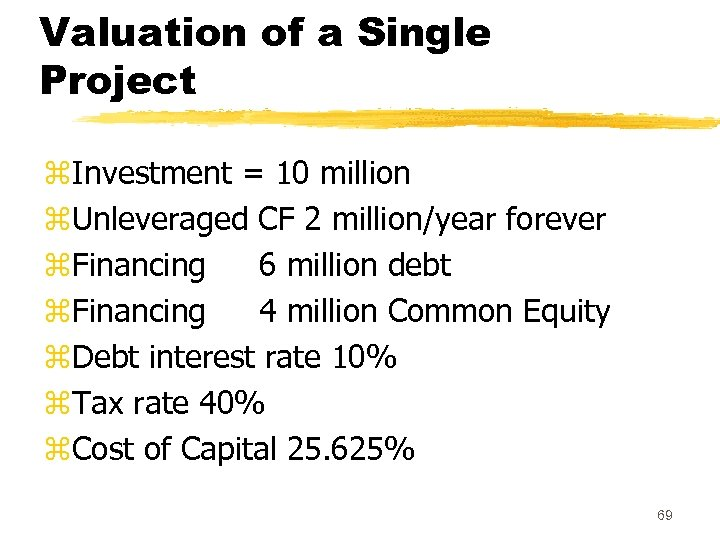Valuation of a Single Project z. Investment = 10 million z. Unleveraged CF 2 million/year forever z. Financing 6 million debt z. Financing 4 million Common Equity z. Debt interest rate 10% z. Tax rate 40% z. Cost of Capital 25. 625% 69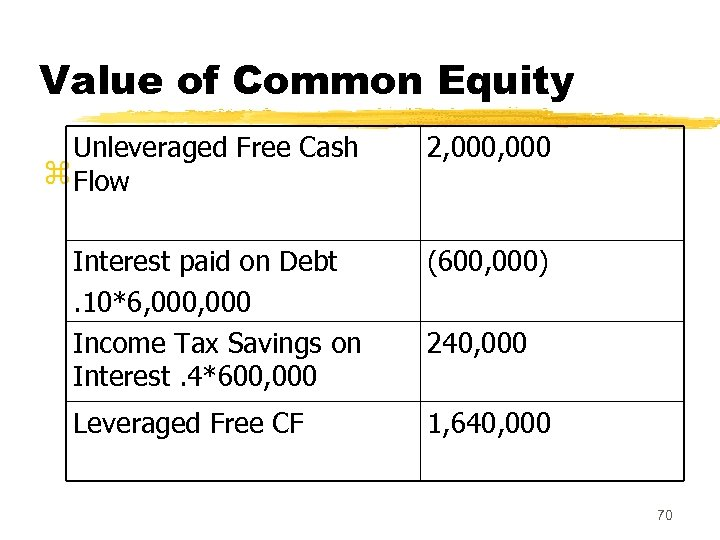Value of Common Equity Unleveraged Free Cash z Flow 2, 000 Interest paid on Debt. 10*6, 000 Income Tax Savings on Interest. 4*600, 000 (600, 000) Leveraged Free CF 1, 640, 000 240, 000 70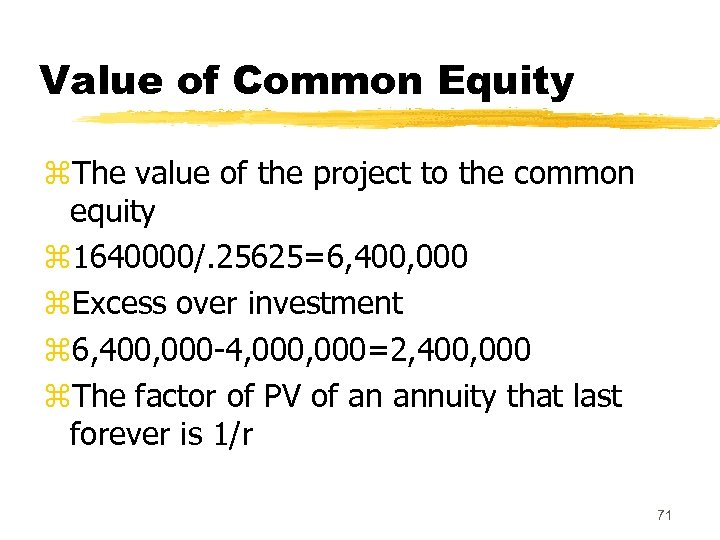Value of Common Equity z. The value of the project to the common equity z 1640000/. 25625=6, 400, 000 z. Excess over investment z 6, 400, 000 -4, 000=2, 400, 000 z. The factor of PV of an annuity that last forever is 1/r 71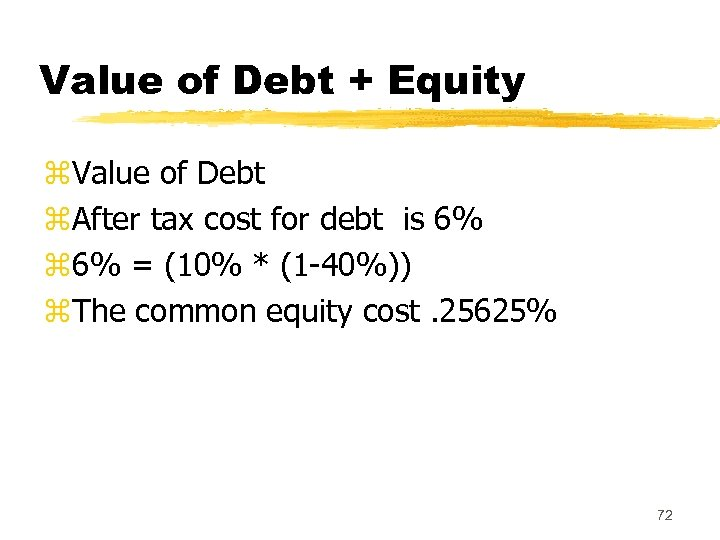Value of Debt + Equity z. Value of Debt z. After tax cost for debt is 6% z 6% = (10% * (1 -40%)) z. The common equity cost. 25625% 72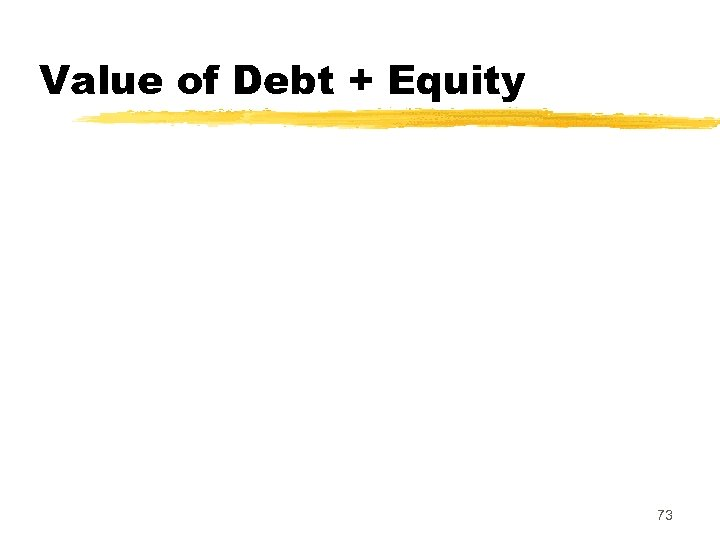Value of Debt + Equity 73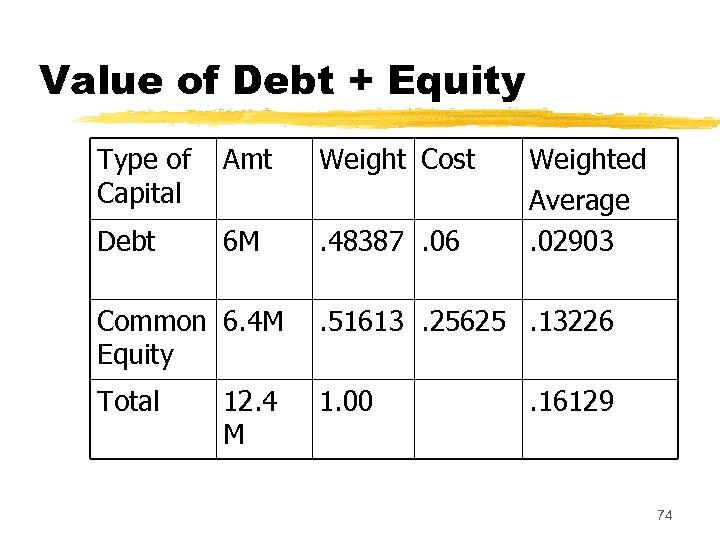Value of Debt + Equity Type of Capital Amt Weight Cost Debt 6 M . 48387. 06 Weighted Average. 02903 Common 6. 4 M Equity . 51613. 25625. 13226 Total 1. 00 12. 4 M . 16129 74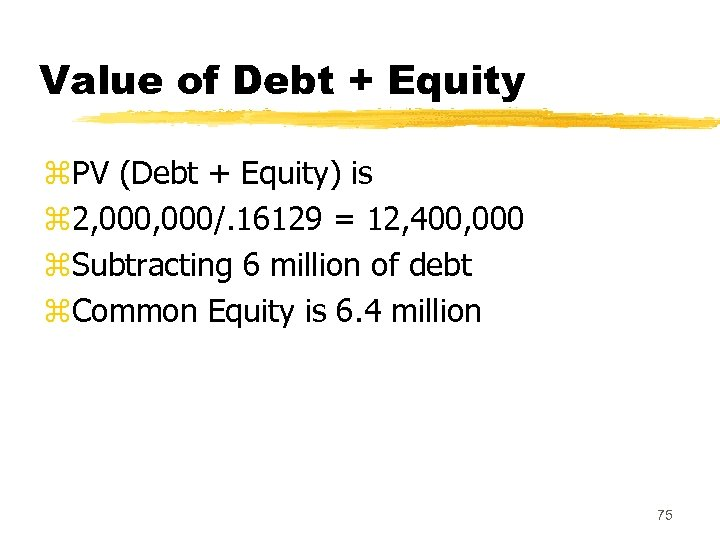Value of Debt + Equity z. PV (Debt + Equity) is z 2, 000/. 16129 = 12, 400, 000 z. Subtracting 6 million of debt z. Common Equity is 6. 4 million 75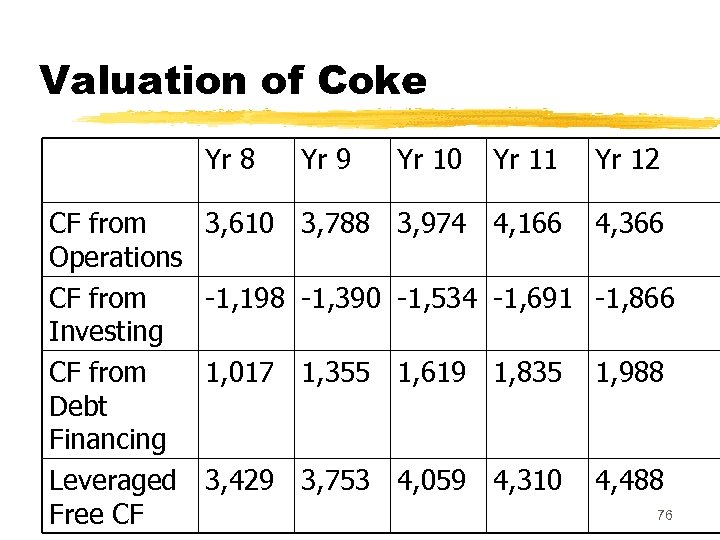Valuation of Coke Yr 8 CF from Operations CF from Investing CF from Debt Financing Leveraged Free CF Yr 9 Yr 10 Yr 11 Yr 12 3, 610 3, 788 3, 974 4, 166 4, 366 -1, 198 -1, 390 -1, 534 -1, 691 -1, 866 1, 017 1, 355 1, 619 1, 835 1, 988 3, 429 3, 753 4, 059 4, 310 4, 488 76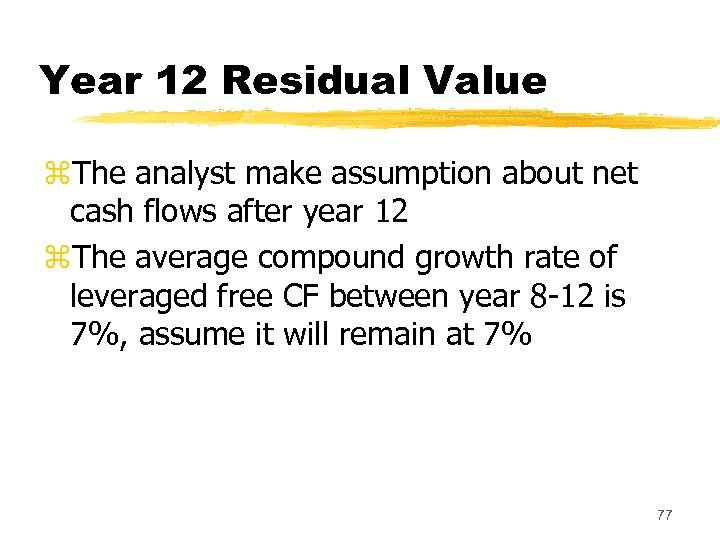Year 12 Residual Value z. The analyst make assumption about net cash flows after year 12 z. The average compound growth rate of leveraged free CF between year 8 -12 is 7%, assume it will remain at 7% 77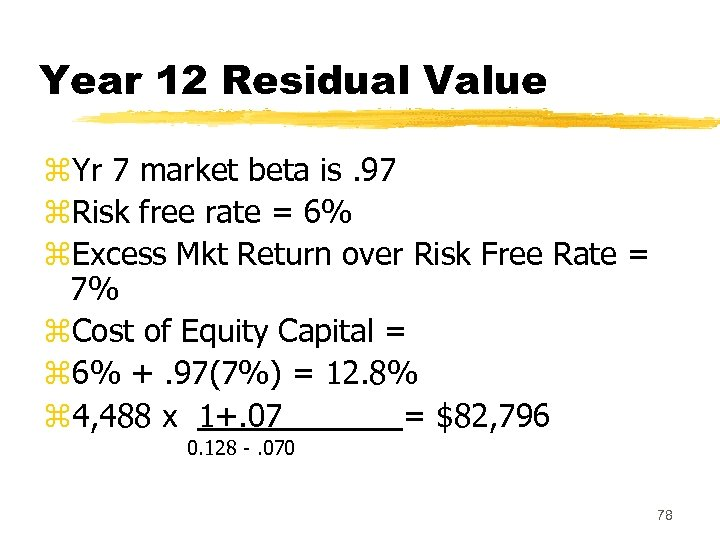Year 12 Residual Value z. Yr 7 market beta is. 97 z. Risk free rate = 6% z. Excess Mkt Return over Risk Free Rate = 7% z. Cost of Equity Capital = z 6% +. 97(7%) = 12. 8% z 4, 488 x 1+. 07 = \$82, 796 0. 128 -. 070 78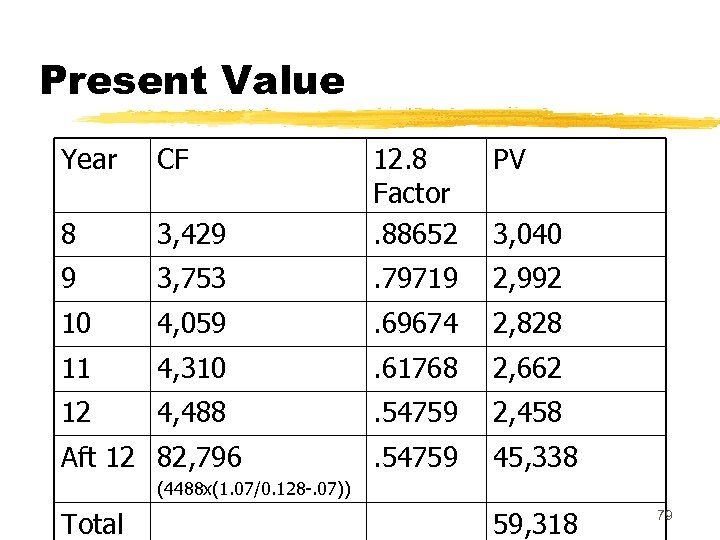Present Value Year CF PV 3, 429 12. 8 Factor. 88652 8 9 3, 753 . 79719 2, 992 10 4, 059 . 69674 2, 828 11 4, 310 . 61768 2, 662 12 4, 488 . 54759 2, 458 . 54759 45, 338 Aft 12 82, 796 3, 040 (4488 x(1. 07/0. 128 -. 07)) Total 59, 318 79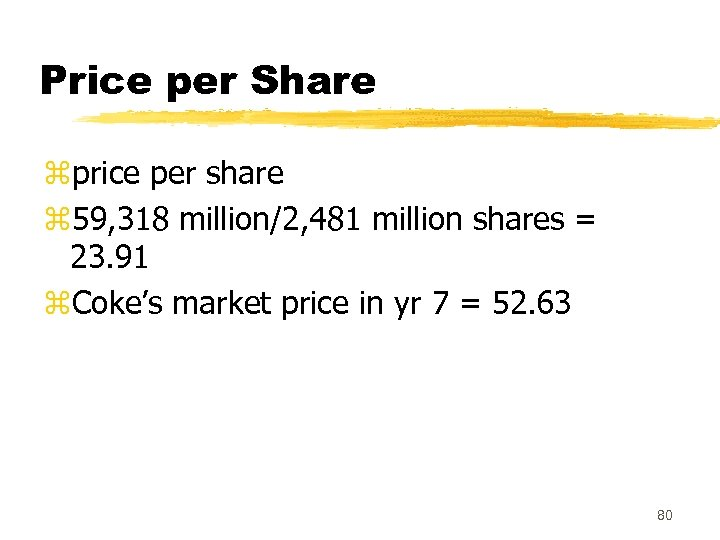Price per Share zprice per share z 59, 318 million/2, 481 million shares = 23. 91 z. Coke’s market price in yr 7 = 52. 63 80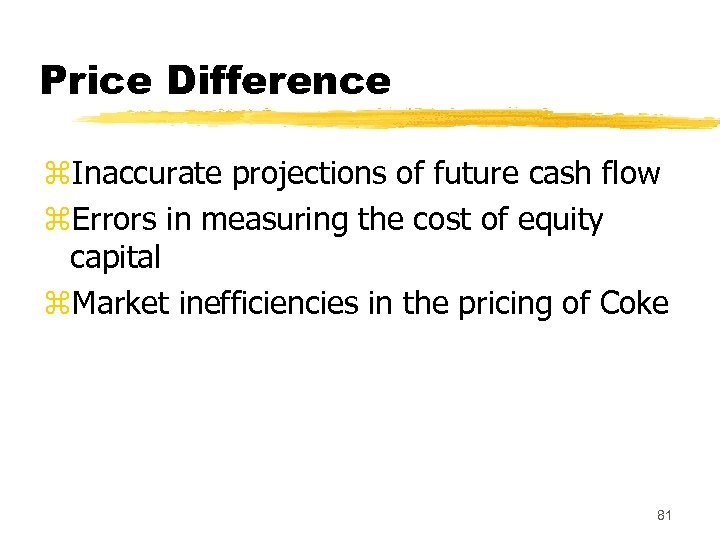Price Difference z. Inaccurate projections of future cash flow z. Errors in measuring the cost of equity capital z. Market inefficiencies in the pricing of Coke 81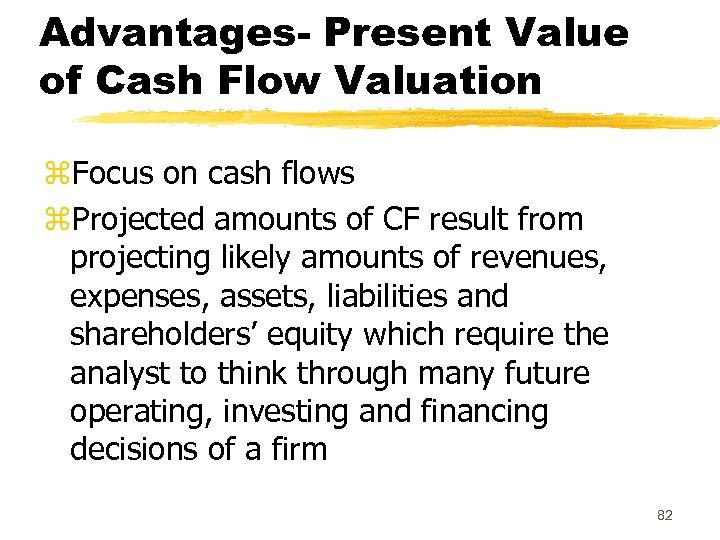Advantages- Present Value of Cash Flow Valuation z. Focus on cash flows z. Projected amounts of CF result from projecting likely amounts of revenues, expenses, assets, liabilities and shareholders’ equity which require the analyst to think through many future operating, investing and financing decisions of a firm 82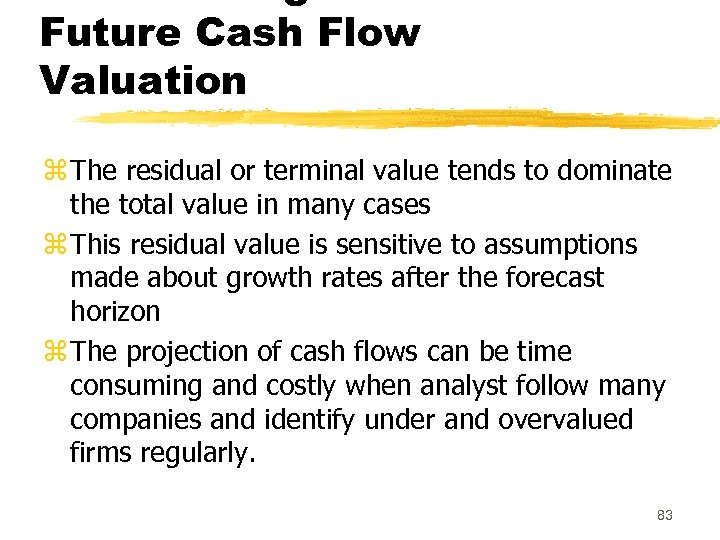Future Cash Flow Valuation z The residual or terminal value tends to dominate the total value in many cases z This residual value is sensitive to assumptions made about growth rates after the forecast horizon z The projection of cash flows can be time consuming and costly when analyst follow many companies and identify under and overvalued firms regularly. 83